[BACK]Computer Modeling in Engineering & SciencesDOI: 10.32604/cmes.2022.019501

ARTICLE

A Personalized Comprehensive Cloud-Based Method for Heterogeneous MAGDM and Application in COVID-19

School of Information Management, Jiangxi University of Finance and Economics, Nanchang, 330013, China
*Corresponding Author: Shuping Wan. Email: shupingwan@163.com
Received: 27 September 2021; Accepted: 30 November 2021

Abstract: This paper proposes a personalized comprehensive cloud-based method for heterogeneous multi-attribute group decision-making (MAGDM), in which the evaluations of alternatives on attributes are represented by LTs (linguistic terms), PLTSs (probabilistic linguistic term sets) and LHFSs (linguistic hesitant fuzzy sets). As an effective tool to describe LTs, cloud model is used to quantify the qualitative evaluations. Firstly, the regulation parameters of entropy and hyper entropy are defined, and they are further incorporated into the transformation process from LTs to clouds for reflecting the different personalities of decision-makers (DMs). To tackle the evaluation information in the form of PLTSs and LHFSs, PLTS and LHFS are transformed into comprehensive cloud of PLTS (C-PLTS) and comprehensive cloud of LHFS (C-LHFS), respectively. Moreover, DMs’ weights are calculated based on the regulation parameters of entropy and hyper entropy. Next, we put forward cloud almost stochastic dominance (CASD) relationship and CASD degree to compare clouds. In addition, by considering three perspectives, a comprehensive tri-objective programing model is constructed to determine the attribute weights. Thereby, a personalized comprehensive cloud-based method is put forward for heterogeneous MAGDM. The validity of the proposed method is demonstrated with a site selection example of emergency medical waste disposal in COVID-19. Finally, sensitivity and comparison analyses are provided to show the effectiveness, stability, flexibility and superiorities of the proposed method.

Keywords: Heterogeneous MAGDM; regulation parameter; C-PLTS; C-LHFS; CASD

1  Introduction

Multi-attribute group decision-making (MAGDM) refers to a decision situation where a group of decision-makers (DMs) provide their own opinions on a given set of alternatives under a set of attributes, and then select the optimal alternative(s) by aggregating their opinions . Since the real-life MAGDM problems often involve multiple different types of attributes, it is not easy for DMs to evaluate all attributes in only one form of evaluation information, which results in the appearance of heterogeneous MAGDM. In heterogeneous MAGDM process, the evaluations of different attributes can be expressed by qualitative and quantitative forms. For example, when a customer selects a car, a real number or an interval number can be used to evaluate its price, but a LT (linguistic term) or its extended forms will be preferred than quantitative value to evaluate its safety. Due to the growing uncertainty of actual decision-making environments, it is more convenient and flexible for DMs to employ qualitative forms, e.g., LT, PLTS (probabilistic linguistic term set), LHFS (linguistic hesitant fuzzy set), to characterize the evaluation information of alternatives on attributes. Both PLTS and LHFS are two important extensions of LT. PLTS, proposed by Pang et al. , consists of LTs and their corresponding probabilities. LHFS, initiated by Meng et al. , contains LTs and their corresponding memberships. For example, a group of DMs are invited to select a site for emergency medical waste disposal during the outbreak of COVID-19. Five attributes, i.e., geographical location, equipment, process technologies, disposal capacity and transport capacity, are chosen to evaluate the alternatives. LTs are suitable to evaluate the geographical location. Since the evaluations for equipment and process technologies are divided into two parts: LTs and corresponding probabilities, PLTSs are suitable to evaluate the equipment and process technologies. Besides, it is easy for DMs to evaluate the disposal capacity and the transportation capacity by using LHFSs. Therefore, the site selection of emergency medical waste disposal is a typical problem of heterogeneous MAGDM with different types of qualitative evaluations. Currently, many scholars have studied heterogeneous MAGDM problems. Yu et al.  developed a fusion method based on trust and behavior analysis for heterogeneous MAGDM scenarios. Liu et al.  proposed a new axiomatic design-based mathematical programming method for heterogeneous MAGDM with linguistic fuzzy truth degrees. Gao et al.  provided a consensus model for heterogeneous MAGDM with several attribute sets. Wan et al.  initiated a new prospect theory based method for heterogeneous MAGDM with hybrid fuzzy truth degrees of alternative comparisons. With the in-depth study of previous literature, many heterogeneous MAGDM problems have been effectively solved. However, there is little research on heterogeneous MAGDM with multiple qualitative forms (especially LT, PLTS and LHFS). To fill the gap, this paper intends to use LTs, PLTSs and LHFSs to portray heterogeneous evaluations.

Qualitative evaluations are not easy to be computed directly, especially when DMs use diverse forms of qualitative evaluations. At present, some models have been developed to deal with the calculations of qualitative evaluations, such as linguistic symbolic model , two-tuple linguistic model , cloud model [14,15]. Linguistic symbolic model and two-tuple linguistic model deal with LTs by converting them into real numbers. Cloud model proposed by Li et al. [14,15] is a more effective tool to describe qualitative concepts since it has strong power in capturing the fuzziness and randomness of LTs, simultaneously. Based on the probability theory and fuzzy set theory, the cloud model utilizes three numerical characteristics, i.e., mathematical expectation Ex, entropy En and hyper entropy He, to realize the nimble and effective inter-transformation between qualitative evaluations and quantitative values. Cloud model has attracted extensive attention from scholars and has been successfully applied to various fields, such as behavioral analysis , artificial intelligence [17,18], system assessment , data mining , knowledge discovery  and decision-making , etc.

Although the above mentioned cloud-based methods  are efficient in handing various practical decision-making problems, there still exist some defects as follows:

(1)   Some previous studies [2227,29,31,32] depicted the evaluations only with a single qualitative form, which might limit their applications in practical decision-making problems.

(2)   Few studies took DMs’ personalities into account during the transformation process. Wang et al.  introduced overlap parameter into the transformation process to reflect the DMs’ personality and preference. But the determination of overlap parameter is a little subjective, which may lead to unreasonable decision results.

(3)   The comprehensive clouds in existing approaches [23,32] may cause the loss and distortion of evaluation information.

(4)   Methods in [22,24,26,29,32] used the expected score values of clouds to rank the alternatives, while methods in [23,27,30,33,34] utilized the closeness coefficient and priority vector to rank the alternatives. However, the expected score values of clouds sometimes are unstable since the expected score values are generated randomly. The closeness coefficient and priority vector depend on the distances between clouds, but different definitions of distance between clouds usually generate different ranking results.

To overcome the above limitations, this paper develops a personalized comprehensive cloud-based method for heterogeneous MAGDM, in which the evaluations of alternatives on attributes are represented as LTs, PLTSs and LHFSs. Regulation parameters of entropy and hyper entropy are proposed to reflect the DMs’ personalities. Two approaches are put forward to transform PLTS and LHFS into comprehensive cloud of PLTS (C-PLTS) and comprehensive cloud of LHFS (C-LHFS), respectively. The cloud almost stochastic dominance (CASD) relationship and CASD degree are initiated to compare clouds and further rank the alternatives. In addition, a novel approach is presented to obtain DMs’ weights and a comprehensive tri-objective programing model is constructed to determine the attribute weights. The proposed method is employed to the site selection of emergency medical waste disposal in COVID-19. Compared with existing studies, the major contributions of this paper are highlighted in the following four aspects:

(1)   Regulation parameters of entropy and hyper entropy are defined objectively. By incorporating regulation parameters into the transformation process, DMs’ personalities are reflected well. Moreover, DMs’ weights are objectively determined based on the proposed regulation parameters.

(2)   From the perspectives of probability and membership degree, two approaches are put forward to transform PLTS and LHFS into C-PLTS and C-LHFS, respectively. The modified ratios of LTs decrease the loss and distortion of evaluation information.

(3)   CASD relationship and CASD degree are defined and used to compare clouds. Based on the proposed comparison approach for clouds, the alternatives are ranked and the ranking results are stable and effective.

(4)   A comprehensive tri-objective programing model is constructed to determine the attribute weights. In this model, three perspectives are considered, including differentiation between evaluation values, relationship between attributes and the amount of information contained in evaluation values. The setting of balance coefficients enables DMs to make a tradeoff in the three perspectives, which can improve the flexibility of the proposed method.

The remainder of this paper is organized as follows: Section 2 briefly introduces some concepts related to LTs and reviews cloud model as well as almost first-degree stochastic dominance (AFSD). Section 3 describes the heterogeneous MAGDM problem and develops two novel transformation approaches from PLTS and LHFS to comprehensive clouds. In Section 4, a personalized comprehensive cloud-based method is proposed for heterogeneous MAGDM problem. A numerical example and sensitivity analyses are conducted to illustrate the proposed method in Section 5. Section 6 performs some comparison analyses to explain the superiorities of the proposed method. Some conclusions are summarized in Section 7.

2  Preliminaries

This section briefly introduces some concepts related to LTs and reviews cloud model as well as AFSD.

2.1 LT and Some Related Concepts

Let S={si|i=1,2,,2τ+1} be a finite and completely ordered discrete term set with odd cardinality , where τ is a nonnegative integer, and si represents a possible value for a LT. The set S is a linguistic term set (LTS) if si,sjS satisfy the following properties:

(i)  Ordered set: sisj if and only if ij;

(ii)  Negation operation: neg(si)=sj, if i+j=2τ+1.

In linguistic evaluation scales, the absolute deviation of semantics between any two adjacent LTs may increase, decrease or remain unchanged with increasing linguistic subscripts. To reflect various semantics deviation, linguistic scale functions (LSFs)  are used to flexibly portray evaluation scales according to specific semantic situations.

Definition 1. [22,36] Let siS be a LT. When θi[0,1] is a numerical value, the LSF is mapped from si to θi(i=1,2,,2τ+1) as follows:

F:siθi,(i=1,2,,2τ+1)

where 0θ1<θ2<<θ2τ+11. θi represents the evaluation of DM when he/she chooses the LT si. As a result, the function F describes the semantics of si(i=1,2,,2τ+1). LSFs are strictly monotonously increasing with respect to the subscript i.

Three kinds of LSFs are shown below:

LSF1: F(si)=θi=i12τ,(i=1,2,,2τ+1)(1)

In LFS1, the absolute deviation between adjacent LTs remains unchanged with increasing linguistic subscripts. Take τ=3 as an example, and the LTs are graphically shown in Fig. 1.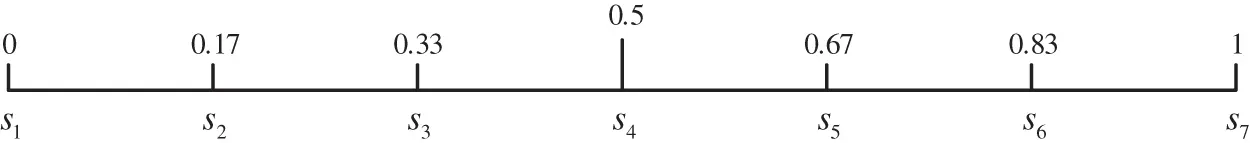Figure 1: LSF1 (τ=3)

LSF2: F(si)=θi={aτaτi+12(aτ1),(i=1,2,,τ+1)aτ+aiτ122(aτ1),(i=τ+2,τ+3,,2τ+1).(2)

Lots of experimental studies  have illustrated that a generally lies in the interval [1.36,1.4]. Moreover, a also can be determined by a subjective approach . In LFS2, the absolute deviation between adjacent LTs gradually increases from the middle of the given LTs to both ends. If we take τ=3 and set a=1.36, the LTs are graphically shown in Fig. 2.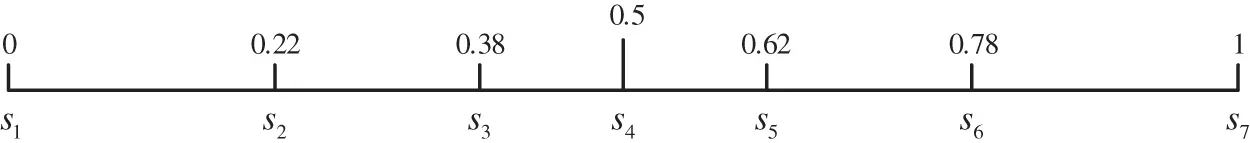Figure 2: LSF2 (τ=3, a=1.36)

LSF3: F(si)=θi={τα(τi+1)α2τα,(i=1,2,,τ+1)τβ+(iτ1)β2τβ,(i=τ+2,τ+3,,2τ+1).(3)

LSF3 is defined based on prospect theory's value function and the DMs’ different sensation for the absolute deviation between adjacent linguistic subscripts. α and β(α,β[0,1]) represent the curvature of the subjective value function for gain and loss, respectively . LSF3 reduces to LSF1 when α=β=1. The absolute deviation between adjacent LTs gradually decreases from the middle of the given LTs to both ends. If we take τ=3 and set α=β=0.8, the LTs are graphically shown in Fig. 3.Figure 3: LSF3 (τ=3, α=β=0.8)

In order to save all of the given information and facilitate calculation, the aforesaid functions can be extended into F:S~R+, where F(si)=θi is a continuous and strictly monotonously increasing function.

Definition 2.  Let S={si|i=1,2,,2τ+1} be a LTS. A PLTS L(p) can be defined as L(p)={s(l)(p(l))|s(l)S,p(l)0,i=1,2,,L(p),l=1L(p)p(l)1}, where s(l)(p(l)) is the LT s(l) associated with the probability p(l), and L(p) denotes the number of all different LTs in L(p). If l=1L(p)p(l)<1, then p~(l)=p(l)/l=1L(p)p(l) is used to normalize the PLTS.

In this paper, it is assumed that all PLTSs have already been normalized.

Definition 3.  Let S={si|i=1,2,,2τ+1} be a LTS. A LHFS LH in S is defined as LH={(s(l),lh(s(l)))|s(l)S}, where lh(s(l))={r1,r2,,rlh(s(l))} is a set with lh(s(l)) values in (0, 1] and denotes the possible membership degrees of the element s(l)S to the set LH. #LH denotes the number of all different LTs in LH, and lh(s(l)) represents the count of real numbers in lh(s(l)).

2.2 Cloud Model

Definition 4.  Let U be the universe of discourse and T be a qualitative concept in U. If xU is a random instantiation of concept T that satisfies xN(Ex,En2) and EnN(En,He2), and y[0,1] is the certainty degree of x belonging to T that satisfies y=exp((xEx)22(En)2), then the distribution of x in the universe U is defined as a normal cloud, and (x,y) represents a cloud drop.

For simplicity, normal cloud is called as cloud hereafter. The degree of certainty of x belonging to concept T is a probability distribution rather than a fixed number. Hence, xU, y=exp((xEx)22(En)2) is a one-to-many mapping.

There are two kinds of uncertainty: randomness and fuzziness. Randomness refers to the uncertainty contained in an event that has a clear definition but do not necessarily occur. Fuzziness refers to the uncertainty contained in an event that has appeared but it is difficult to define it accurately . There is a practical demand to describe fuzziness and randomness inherent in LTs simultaneously. Cloud can perfectly depict the overall quantitative properties of a concept through three numerical characteristics: mathematical expectation Ex, entropy En and hyper entropy He, where Ex is the mathematical expectation of cloud drops belonging to a concept in the universe, and En reflects the uncertainty measurement of a qualitative concept, including randomness and fuzziness. From the perspective of probability theory, En is similar to standard variance of random variables. From the point of fuzzy set theory, En represents the scope in which cloud drops are accepted by the concept, and it indicates the support set of the concept with membership degrees larger than 0. As a result, En reflects randomness and fuzziness of a qualitative concept and their correlation, simultaneously. He represents the degree of uncertainty of En, i.e., the second-order entropy of the entropy [15,40]. A cloud can be described by Ex, En, He, and denoted by C=(Ex,En,He).

Definition 5.  Given two clouds C1=(Ex1,En1,He1) and C2=(Ex2,En2,He2), some operations of clouds are defined as follows:

(1)   C1+C2=(Ex1+Ex2,En12+En22,He12+He22);

(2)   C1C2=(Ex1Ex2,En12+En22,He12+He22);

(3)   γC1=(γEx1,γEn1,γHe1), (γ0).

2.3 AFSD

The AFSD is used to compare two stochastic variables. It was proposed by Leshno and Levy . Let X1 and X2 be two stochastic variables, where G1(x) and G2(x) denote two cumulative distribution functions, respectively. Let Ω={x|G1(x)>G2(x)}, Θ={x|G2(x)>G1(x)} and ||G1(x)G2(x)||=ΩG1(x)G2(x)dx+ΘG2(x)G1(x)dx. Then, AFSD is defined below:

Definition 6. [41,42] For 0<δ<0.5, X1 dominates X2 by δAFSD if and only if ΩG1(x)G2(x)dxδ||G1(x)G2(x)||, where ||G1(x)G2(x)|| corresponds to the area between G1 and G2, ΩG1(x)G2(x)dx corresponds to the area that G1 is greater than G2, and δ denotes the degree of first-degree stochastic dominance violation allowed.

3  Heterogeneous MAGDM Problem and Comprehensive Cloud

This section describes the heterogeneous MAGDM problem and introduces the improved transformation approach between LT and cloud in detail. Particularly, we developed two novel transformation approaches from PLTS and LHFS to comprehensive clouds.

3.1 Description for Heterogeneous MAGDM Problem

A heterogeneous MAGDM problem is to find the best solution from all feasible alternatives assessed on multiple attributes by a group of DMs. The evaluation attributes in heterogeneous MAGDM can be classed into several subsets which are expressed by different kinds of forms.

For a heterogeneous MAGDM problem, suppose that DMs de(e=1,2,,k) have to select the optimal alternative(s) from a group of alternatives xu(u=1,2,m) or rank these alternatives based on attributes yv(v=1,2,n). Denote an alternative set by X={x1,x2,,xm}, an attribute set by Y={y1,y2,,yn}, and a DM set by D={d1,d2,,dk}. Denote Y1={y1,y2,,yv1},Y2={yv1+1,yv1+2,,yv2}, Y3={yv2+1,yv2+2,,yv3}, respectively, where 1v1v2v3n. Namely, Y is divided into three subsets Yt(t=1,2,3), where Yt(t=1,2,3) are attribute subsets in which attribute values are expressed with LTs, PLTSs and LHFSs respectively. YtYl=(t,l=1,2,3;tl), t=13Yt=Y, where is an empty set. Denote M={1,2,,m}, N1={1,2,,v1},N2={v1+1,v1+2,,v2}, N3={v2+1,v2+2,,n}, N={1,2,,n} and K={1,2,,k}. Denote the DM weight vector by v=(ϖ1,ϖ2,,ϖk)T, where ϖe is the weight of DM de, satisfying that 0ϖe1(e=1,2,,k) and e=1kϖe=1. Denote the attribute weight vector by w=(w1,w2,,wn)T, where wv is the weight of attribute yv, satisfying that 0wv1(v=1,2,,n) and v=1nwv=1 .

Let ruve be the evaluation of an alternative xu on attribute yv given by DM de. If vN1, ruve is a LT, denoted by si(siS); If vN2, ruve is a PLTS, denoted by L(p)={s(l)(p(l))|s(l)S}; If vN3, ruve is a LHFS, denoted by LH={(s(l),lh(s(l)))|s(l)S}. After normalizing, the individual original normalized evaluation matrix Re=(ruve)m×n can be obtained as

Re=(ruve)m×n=(ruve)m×n=y1y2ynx1x2xm[r11er12er1ner21er22er2nerm1erm2ermne](e=1,2,,k)(4)

3.2 Transformation between LT and Cloud

Generally, two kinds of approaches have been proposed for transformation from LTs to clouds so far. One is based on the golden radio , and the other is based on the LSF . Wang et al.  introduced a parameter named overlapping degree into the transformation approach  to determining the degree of overlap between two adjacent clouds. With the parameter ε[εmin,εmax], DMs could express their preference for the degree of overlap between two adjacent clouds. However, after processing the calculation formulae for three numerical characteristics, a problem emerges. That is, once the LSF and some related parameters are fixed in , D(Exi,i+1)=Exi+1Exi3, D(Exi1,i+1)=Exi+1Exi16 and D(Exi1,i)=ExiExi13 are three fixed values. It is easy to see that the values of Eni and Hei are linearly dependent on εmax+εmin2 and εmaxεmin6, respectively. However, the determination of overlapping degree is entirely based on the subjective preference of DMs, which means the determination of Eni and Hei is also subjective. To overcome the above defects, two regulation parameters ς and ζ for entropy and hyper entropy are proposed in this paper. We improve the transformation approach in  by replacing εmax+εmin2 (εmaxεmin6) with ς (ζ) during the transformation process. Significantly, the determination of ς and ζ is totally objective and logical. The specific approaches to determining ς and ζ are stated in Sections 3.2.1 and 3.2.2.

Let S={si|i=1,2,,2τ+1} be a LTS, L(p)={s(l)(p(l))|s(l)S} be a normalized PLTS, and LH={(s(l),lh(s(l)))|s(l)S} be a LHFS. L(p)uve={suv(l)e(puv(l)e)} denotes the evaluation of an alternative xu on attribute yv(vN2) given by the DM de, and L(p)uve[1,2τ+1] denotes the number of all different LTs in L(p)uve. LHuve={(s(l),lh(s(l))uve)|s(l)S} indicates the evaluation of an alternative xu on attribute yv(vN3) given by the DM de. #LHuve[1,2τ+1] signifies the number of all different LTs in LHuve. #lh(s(l))uve represents the count of real numbers in lh(s(l))uve={ruv,1e,ruv,2e,,ruv,#lh(s(l))uvee}. lh(s(l))uve is a set with #lh(s(l))uve values in (0, 1] and denotes the possible membership degrees of the element s(l)S to the set LHuve.

3.2.1 Determination of the Regulation Parameter of Entropy

Entropy En reflects the uncertainty measurement of a qualitative concept, specifically randomness and fuzziness. From the perspective of fuzzy set theory, it represents the scope in which the cloud drops are accepted by the concept. It is common that the more elements are used in evaluations, the more hesitant the DM is. Hence, for a hesitant DM, larger En should to be assigned to its LTs. Therefore, the regulation parameter of En should be determined according to DMs’ hesitant degree.

Definition 7. For a heterogeneous MAGDM, the average number of LTs used by DM de at an evaluation in the form of PLTSs or LHFSs, is defined as

ηe=1m(nv1)u=1m[v=v1+1v2#L(p)uve+v=v2+1n#LHuve].(5)

It is obvious that ηe[1,2τ+1].

Definition 8. The hesitant degree of DM de is defined as

HDe=ηe/(2τ+1).(6)

Obviously, HDe[12τ+1,1].

The determination of DM's hesitant degree is based on the average number of LTs that DM uses at a single evaluation in the form of PLTSs or LHFSs.

A great deal of experimental research has demonstrated that regulation parameter ς generally lies in [1,2]. In fact, if the hesitant degree HDe is 12τ+1, it means that only one LT is used by DM de at each evaluation in the form of PLTSs or LHFSs. In this situation, DM de is regarded as a decisive and confident person. Accordingly, it is appropriate for DM de to take 1 as the value of ς. The more the LTs used by DM de, the bigger the value of ς is. Based on this premise, the regulation parameter ς of entropy is defined as follows:

Definition 9. Let ρ1=1+2τ2τ+1. The regulation parameter of entropy for DM de is defined as

ςe=logρ1(ηe2τ+1+2τ2τ+1)+1=logρ1(HDe+2τ2τ+1)+1,(7)

where ηe[1,2τ+1], HDe[12τ+1,1]. Clearly, it holds that ςe[1,2].

In the following, an example is given to illustrate how to determine the value of ς.

Example 1. Let S={si|i=1,2,,7} be a LTS. There are three alternatives x1, x2 and x3. In order to select the optimal alternative, DM de gives evaluations for x1, x2, x3 on three attributes y1, y2, y3. The evaluations for y1, y2 and y3 are expressed in the forms of LT, PLTS and LHFS, respectively. DM de gives an evaluation matrix as follows:

Re=(ruve)3×3=y1y2y3x1x2x3[s4s6(0.1),s7(0.9)(s3,0.8,0.9),(s4,0.6)s2s3(0.1),s4(0.2),s5(0.7)(s5,0.7)s6s4(1)(s1,0.8) ]

Calculate the average number of LTs used by DM de at an evaluation in the form of PLTSs or LHFSs by Eq. (5):

ηe=13×2×[(2+2)+(3+1)+(1+1)]=1.6667

Calculate the hesitant degree of DM de by Eq. (6):

HDe=1.66677=0.2381

Then, the value of ςe is calculated by Eq. (7):

ςe=log1+0.8571(0.2381+0.8571)+1=1.1470

3.2.2 Determination of the Regulation Parameter of Hyper Entropy

Hyper entropy He represents the degree of uncertainty of En, i.e., the second-order entropy of the entropy. The larger He is, the thicker the cloud is, and the wider the distribution of membership is. Thus, on the one hand, the information entropy as a very important concept to measure the uncertainty in evaluation information provided by DMs, the larger it is, the larger He should be. On the other hand, membership degree as an index to measure the degree that an element belongs to a certain concept, the lower it is, the larger the indeterminacy degree is. Furthermore, the larger indeterminacy degree in the decision matrix provided by DM, the larger He should to be. With the above analysis, the regulation parameter of He should be determined according to indeterminacy degree and information entropy.

Definition 10.  The information entropy of L(p) is defined as follows:

H(L(p))=log2zl=1#L(p)(p(l))log2(p(l)),(8)

where z is a constant that is set to 1.28 in this paper as  sets. It is easily seen that H(L(p))[0,log2zlog2(2τ+1)].

Definition 11. The information entropy of DM de is defined as follows:

He=log2zm(v2v1)u=1mv=v1+1v2l=1L(p)uve(p(l))log2(p(l)),(9)

where z is a constant that is set to 1.28 in this paper as  sets. Obviously, He[0,log2zlog2(2τ+1)].

The closer the memberships for corresponding LTs are to 0, the larger indeterminacy degree DMs have for corresponding LT.

Definition 12. The indeterminacy degree of LH={(s(l),lh(s(l)))|s(l)S} is defined as follows:

ID(LH)=1#LHl=1#LH[1#lh(s(l))rlh(s(l))(1r)].(10)

Clearly, it holds that ID(LH)[0,1).

Definition 13. The indeterminacy degree of DM de is defined as follows:

IDe=1m(nv2)u=1mv=v2+1n{1#LHuvel=1#LHuve[1#lh(s(l))uveruvelh(s(l))uve(1ruve)]}.(11)

It is easily seen that IDe[0,1).

Definition 14. Let ρ2=1+log2zlog2(2τ+1). The regulation parameter of hyper entropy for DM de is defined as

ζe=12[logρ2(He+1)+log2(IDe+1)],(12)

where He[0,log2zlog2(2τ+1)], IDe[0,1). Obviously, ζe[0,1).

Take τ=3 as an example. The graphical representation for the regulation parameter of hyper entropy is shown in Fig. 4.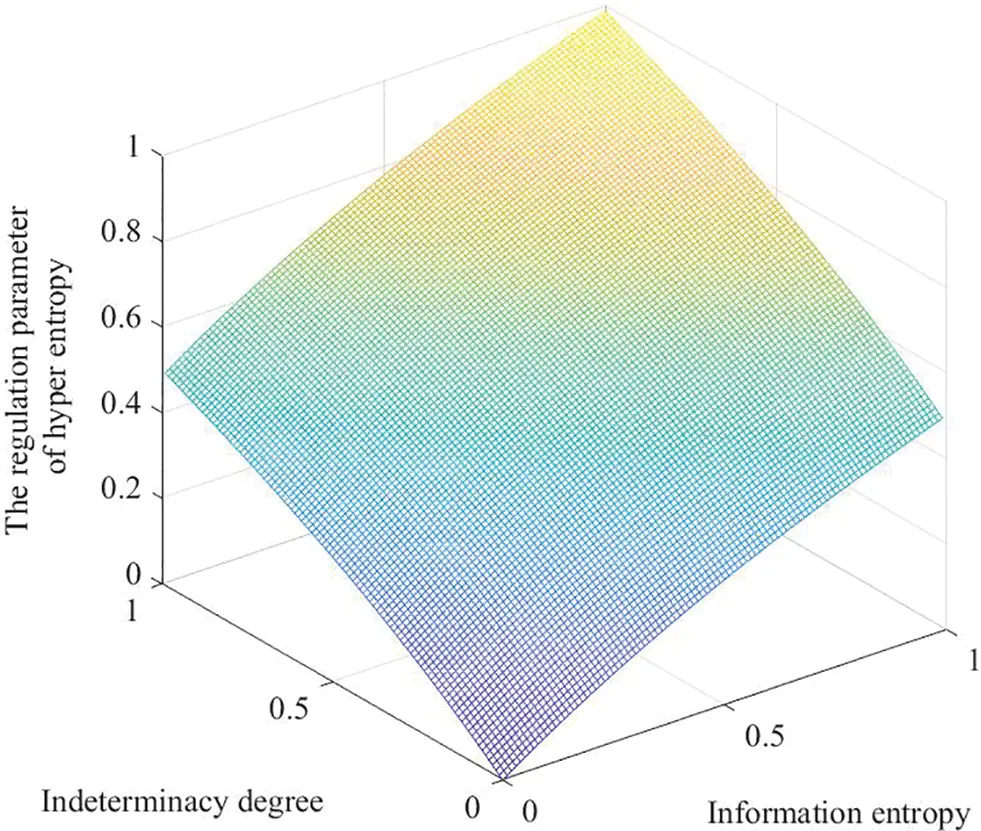Figure 4: Graphical representation for the regulation parameter of hyper entropy (τ=3)

Example 2. Following Example 1, the value of ζe can be calculated as follows:

Calculate the information entropy of each evaluation in the form of PLTSs by Eq. (8):

H({s6(0.1),s7(0.9)})=log21.28×(0.1×log20.1+0.9×log20.9)=0.1670,

H({s3(0.1),s4(0.2),s5(0.7)})=log21.28×(0.1×log20.1+0.2×log20.2+0.7×log20.7)=0.4120,

H({s4(1)})=log21.28×(1×log21)=0

Calculate the information entropy of DM de by Eq. (9):

He=13×(0.1670+0.4120+0)=0.1930

Calculate the indeterminacy degree of each evaluation in the form of LHFSs by Eq. (10):

ID({s3(0.8,0.9),s4(0.6)})=12×{12×[(10.8)+(10.9)]+(10.6)}=0.275,

ID({s5(0.7)})=10.7=0.3,

ID({s1(0.8)})=10.8=0.2

Calculate the indeterminacy degree of DM de by Eq. (11):

IDe=13×(0.275+0.3+0.2)=0.2583

Then, the value of ζe is calculated by Eq. (12):

ζe=12×[log1.9998(0.1930+1)+log2(0.2583+1)]=0.2931

3.2.3 Specific Procedures for Transformation between LT and Cloud

Definition 15.  Let S={si|i=1,2,,2τ+1} be a LTS, where τ is a positive integer. A valid universe [Xmin,Xmax] is provided by DMs. Then, a LT si(i=1,2,,2τ+1) can be represented by the normal cloud Ci=(Exi,Eni,Hei).

Then, the specific transformation procedures are shown as follows:

(1)   Calculate ς and ζ.

Determination approaches for ς and ζ are shown in Sections 3.2.1 and 3.2.2.

(2)   Calculate θi.

Map si to θi using LSFs.

LSF2 Eq. (2) is adopted in this paper, where a=1.36.

(3)   Calculate Exi.

Exi=Xmin+θi(XmaxXmin).(13)

(4)   Calculate Eni.

Let (x,y) be a cloud drop, where xN(Ex,En2). According to 3σ principle of the normal distribution, 99.7% cloud drops of Ci should be located in the interval [Exi1,Exi+1]. However, since the distances between Exi and Exi1 are different from the distances between Exi and Exi+1, the entropy of Ci(i=2,3,,2τ) are different for the right side and the left side. For simplicity, Eni(i=2,3,,2τ) take the mean value of ςEni_ and ςEni¯.

Eni={ςEni¯=ςExi+1Exi3,i=1ςEni¯+Eni_2=ςExi+1Exi16,2i2τςEni_=ςExiExi13,i=2τ+1,(14)

where ςEni_=ςExiExi13, ςEni¯=ςExi+1Exi3, ςEni_ denotes Eni for the left side, and ςEni¯ denotes Eni for the right side.

(5)   Calculate Hei

Hei={ζExi+1Exi3,i=1ζExi+1Exi16,2i2τζExiExi13,i=2τ+1.(15)

Based on the above analyses, the corresponding cloud for LT si can be generated by Algorithm 1.To illustrate the advantages of regulation parameters, an example is given below.

Example 3. Given a universe [Xmin,Xmax] and a LTS S={si|i=1,2,,7}. For alternatives x1, x2, x3 regarding three attributes y1, y2, y3, DMs d1, d2 and d3 give their evaluations. The evaluations for y1, y2 and y3 are expressed in the forms of LT, PLTS and LHFS, respectively. DMs d1, d2 and d3 give their evaluation matrices as follows:

R1=(ruv1)3×3=y1y2y3x1x2x3[ s4s6(1)(s3,0.9)s2s3(1)(s5,1)s6s4(1)(s1,0.95) ]

R2=(ruv2)3×3=y1y2y3x1x2x3[s4s6(0.1),s7(0.9)(s3,0.8,0.9),(s4,0.6)s2s3(0.1),s4(0.2),s5(0.7)(s5,0.7)s6s4(1)(s1,0.8) ]

R3=(ruv3)3×3=y1y2y3x1x2x3[s4s6(0.5),s7(0.5)(s3,0.2),(s4,0.1)s2s3(0.3),s4(0.3),s5(0.4)(s5,0.2)s6s4(1)(s1,0.1) ]

Based on Eqs. (5)(12), the regulation parameters ς and ζ for each DM are calculated as follows:

ς1=1,ζ1=0.0352,ς2=1.1470,ζ2=0.2931,ς3=1.1470,ζ3=0.6359;

Based on Eqs. (2) and (13)(15), θi, Exi, Eni, Hei(i=1,2,,7) for DMs d1, d2, d3 are calculated and the results are shown in Tables 13, respectively.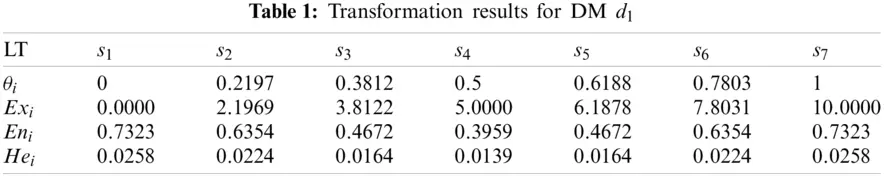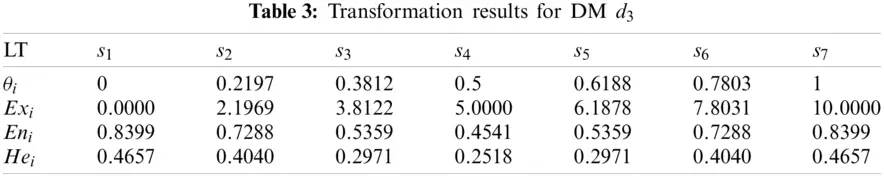Three sets of cloud generated by DMs d1, d2, d3 are graphically shown in Figs. 57, respectively.

It can be seen from Figs. 57 that the cloud drops distribution varies in width and thickness for different DMs. In previous studies [22,23,2531,46], clouds for the corresponding LTS are usually the same for all DMs. However, since different DMs have different personalities, knowledge and experience, the width and thickness of clouds for the corresponding LTS should be different for different DMs. The more sure, confident and decisive the DM is for its evaluation matrix, the more concentrated and thinner the cloud distribution should be, vice versa. Unfortunately, most previous studies failed to notice this characteristic, whereas this paper sufficiently takes this characteristic into account. Although the overlap degree is considered in  to obtain personalized cloud sets for DMs, the determination of overlap degree depends on DMs’ subjective intuition. This defect is overcome in this paper. The regulation parameters are determined according to the evaluation matrix given by DM, which means the determination of regulation parameters is objective and logical.Figure 5: Clouds for the LTs used by d1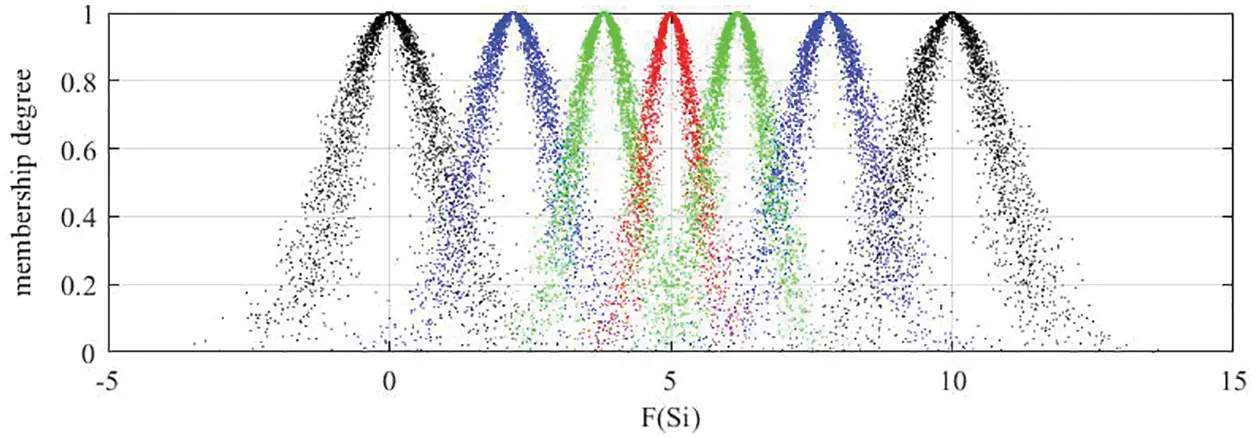Figure 6: Clouds for the LTs used by d2Figure 7: Clouds for the LTs used by d3

3.3 Transformation from PLTS and LHFS to Comprehensive Clouds

In this sub-section, two approaches are brought forward to transform PLTS and LHFS into comprehensive clouds, respectively.

3.3.1 Transformation from PLTS to Comprehensive Cloud

Definition 16. Let S={si|i=1,2,,2τ+1} be a LTS. The valid universe is [Xmin,Xmax]. Let L(p)={s(l)(p(l))|s(l)S} be a normalized PLTS. The cloud Cs(l)(Exs(l),Ens(l),Hes(l)) represents LT s(l)S. Then, CL(p)(ExL(p),EnL(p),HeL(p)) can be defined as C-PLTS, which is characterized by three numerical characteristics ExL(p), EnL(p) and HeL(p).

Definition 17.  Given a cloud C=(Ex,En,He), if (x,y) is a cloud drop of C, x satisfies xN(Ex,En2) and EnN(En,He2). Then, the normal curve (NC) of all cloud drops can be defined as f=exp((xEx)22En2).

In this paper, g=12πEnexp((xEx)22En2) is utilized to represent the probability density function curve (PDFC) of C.

The specific procedures to determine ExL(p), EnL(p), HeL(p) of C-PLTS are as follows:

(1)   Let Cs(l) be the corresponding clouds for s(l)(l=1,2,,L(p)), Cs(l)(p(l)) be Cs(l) with corresponding probability p(l) and χl,l+1[Exs(l),Exs(l+1)] be the abscissa value of intersection point between the PDFCs of Cs(l)(p(l)) and Cs(l+1)(p(l+1)). If Exs(l)+3Ens(l)>Exs(l+1)3Ens(l+1)(l{1,2,,L(p)1}), then Eq. (16) is used to calculate the value of χl,l+1.

p(l)12πEns(l)exp((χl,l+1Exs(l))22(Ens(l))2)=p(l+1)12πEns(l+1)exp((χl,l+1Exs(l+1))22(Ens(l+1))2),(χl,l+1[Exs(l),Exs(l+1)]).(16)

(2)   Use Eq. (17) to calculate the area for the PDFC of Cs(l)(p(l)) from lower limit to upper limit, which can be denoted by As(l).

As(l)={p(l)12πExs(l)Exs(l)3Ens(l)χl,l+1exp((xExs(l))22(Ens(l))2)dx,l=1p(l)12πExs(l)χl1,lχl,l+1exp((xExs(l))22(Ens(l))2)dx,l{2,3,,L(p)1}p(l)12πExs(l)χl1,lExs(l)+3Ens(l)exp((xExs(l))22(Ens(l))2)dx,l=L(p).(17)

If Exs(l1)+3Ens(l1)Exs(l)3Ens(l)(l{2,3,,L(p)}) (Exs(l)+3Ens(l)Exs(l+1)3Ens(l+1)(l{1,2,,L(p)1})), then Exs(l)3Ens(l) (Exs(l)+3Ens(l)) is substituted for χl1,l (χl,l+1).

(3)   The modified ratio of s(l), denoted by ts(l), will be calculated by Eq. (18):

ts(l)=As(l)l=1#L(p)As(l),(l=1,2,,L(p)).(18)

(4)   According to Definition 5, three numerical characteristics ExL(p), EnL(p), HeL(p) of C-PLTS will be obtained as follows:

ExL(p)=l=1#L(p)(ts(l)Exs(l)),(19)

EnL(p)=l=1#L(p)[ts(l)(Ens(l))2],(20)

HeL(p)=l=1#L(p)[ts(l)(Hes(l))2].(21)

Based on the above analyses, the comprehensive cloud of PLTS L(p)={s(l)(p(l))|s(l)S} can be generated by Algorithm 2.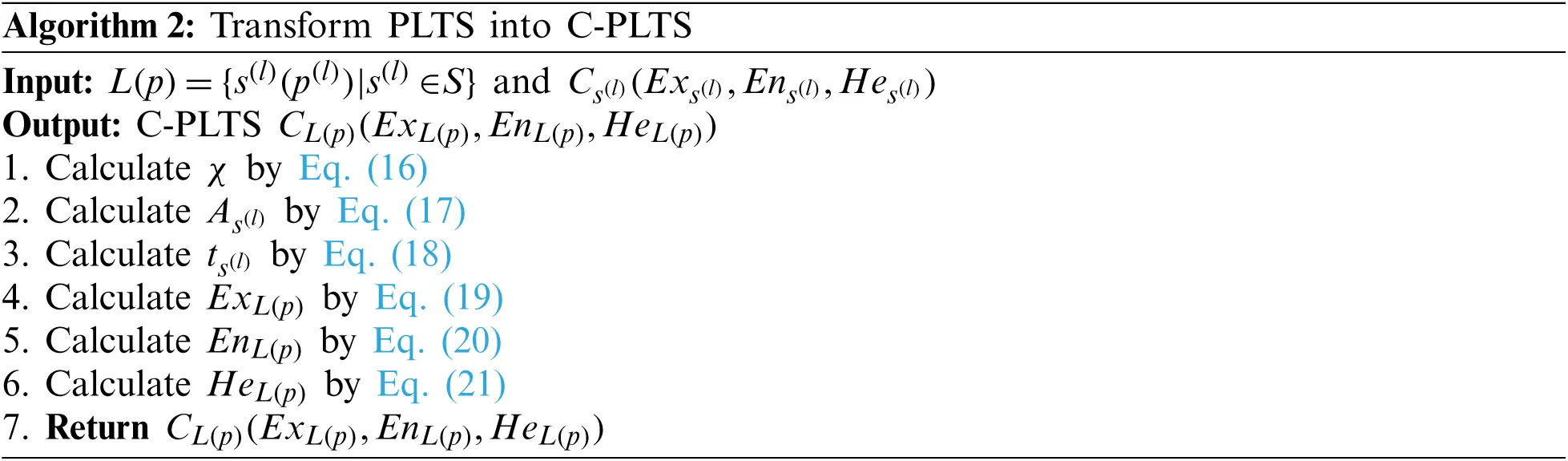Example 4. Given a LTS S={si|i=1,2,,7} and a PLTS L(p)={s4(0.8),s5(0.2)}, C4=(5,0.3959,0.0396) is the corresponding cloud for LT s4 and C5=(6.1878,0.4672,0.0467) is the corresponding cloud for LT s5. The C-PLTS CL(p)(ExL(p),EnL(p),HeL(p)) for L(p)={s4(0.8),s5(0.2)} can be obtained as follows:

(1)   Based on Eq. (16), the abscissa value of the intersection point χ is obtained:

Let 0.8×12π×0.3959×exp((χ4,55)22×0.39592)=0.2×12π×0.4672×exp((χ4,56.1878)22×0.46722) to solve the abscissa value of the intersection point: χ4,5=5.7788.

(2)   Based on Eq. (17), the area for the PDFC of Cs4(0.8) from Ex43En4 to the intersection point and the area for the PDFC of Cs5(0.2) from the intersection point to Ex5+3En5 are obtained:

As4=0.8×12π×0.3959×3.81225.7788exp((x5)22×0.39592)dx=0.7792,As5=0.2×12π×0.4672×5.77887.5893exp((x6.1878)22×0.46722)dx=0.1616

(3)   Based on Eq. (18), the modified ratios of s4 and s5 are obtained:

ts4=0.77920.7792+0.1616=0.8282,ts5=0.16160.7792+0.1616=0.1718

(4)   Based on Eqs. (19)(21), three numerical characteristics are obtained:

ExL(p)=0.8282×5+0.1718×6.1878=5.2040,EnL(p)=0.8282×0.39592+0.1718×0.46722=0.4090HeL(p)=0.8282×0.03962+0.1718×0.04672=0.0409

Finally, the C-PLTS of L(p)={s4(0.8),s5(0.2)} is Cs4(0.8),s5(0.2)}(5.2040,0.4090,0.0409).

The PDFCs and 5000 cloud drops of Cs4(0.8) and Cs5(0.2) are shown in Fig. 8. The areas for the PDFCs of Cs4(0.8) and Cs5(0.2) are shown in Fig. 9.Figure 8: PDFCs and 5000 cloud drops of Cs4(0.8) and Cs5(0.2)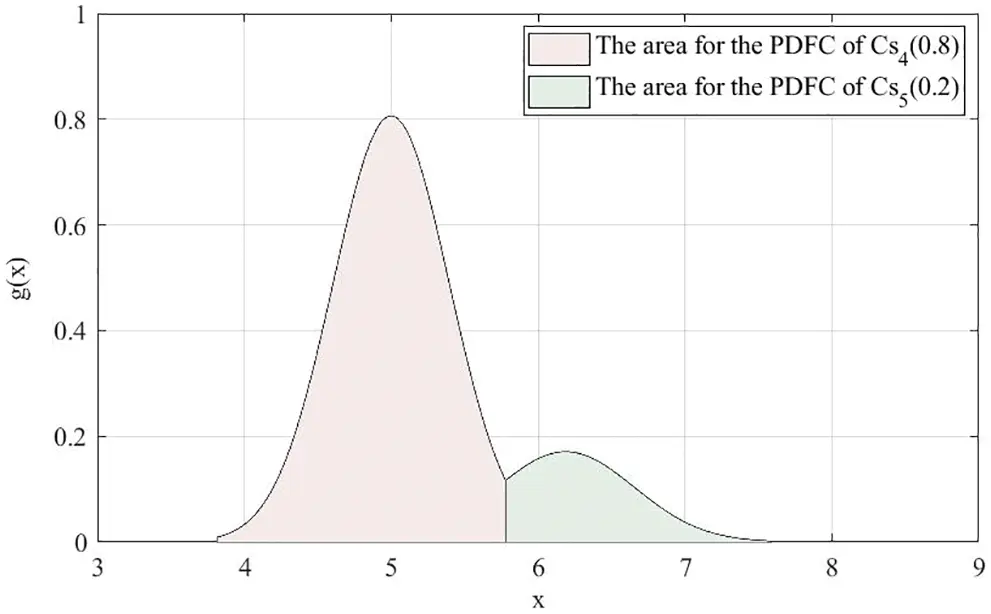Figure 9: Areas for the PDFCs of Cs4(0.8) and Cs5(0.2)

From L(p)={s4(0.8),s5(0.2)}, we can know that the proportions of LTs s4 and s5 are 0.8 and 0.2, respectively. If a DM uses L(p)={s4(0.8),s5(0.2)} to evaluate an alternative, it can be assumed that the DM uses 5000 cloud drops to express his/her opinion, then he/she will place 4000 cloud drops in the s4 region and 1000 cloud drops in s5 region. However, it can be seen from Fig. 8 that parts of the 4000 cloud drops belonging to s4 region will overlap with cloud drops belonging to s5 region, and parts of the 1000 cloud drops belonging to s5 region will overlap with cloud drops belonging to s4 region. In order to eliminate the information distortion caused by the overlapping part, this paper eliminates the overlapped cloud drops from the cloud drops originally allocated and recalculates the proportions that belong to each region. PLTS contains LTs and their corresponding probabilities. Thus the intersection point between the PDFC of Cs4(0.8) and the PDFC of Cs5(0.2) is taken as the boundary to recalculate the proportions of cloud drops distributed in the two regions, which are shown in Fig. 9. From the perspective of probability, the C-PLTS is obtained. In the meanwhile, the modified ratios of LTs decrease the loss and distortion of evaluation information.

3.3.2 Transformation from LHFS to Comprehensive Cloud

Definition 18. Let S={si|i=1,2,,2τ+1} be a LTS. The valid universe is [Xmin,Xmax]. Let LH={(s(l),lh(s(l)))|s(l)S} be a LHFS. The cloud Cs(l)(Exs(l),Ens(l),Hes(l)) represents LT s(l)S. Then, CLH(ExLH,EnLH,HeLH) can be defined as C-LHFS, which is characterized by three numerical characteristics ExLH, EnLH and HeLH.

The specific procedures to determine ExLH, EnLH, HeLH of C-LHFS are as follows:

(1)   Let Cs(l) be the corresponding clouds for s(l)(l=1,2,,LH), C(s(l),lh(s(l))) be Cs(l) with corresponding average value of membership degrees 1#lh(s(l))rlh(s(l))r and χl,l+1[Exs(l),Exs(l+1)] be the abscissa value of intersection point between the NCs of C(s(l),lh(s(l))) and C(s(l+1),lh(s(l+1))). If Exs(l)+3Ens(l)>Exs(l+1)3Ens(l+1)(l{1,2,,LH1}), then Eq. (22) is used to calculate the value of χl,l+1.

(1#lh(s(l))rlh(s(l))r)exp((χl,l+1Exs(l))22(Ens(l))2)=(1#lh(s(l+1))rlh(s(l+1))r)exp((χl,l+1Exs(l+1))22(Ens(l+1))2),(χl,l+1[Exs(l),Exs(l+1)]).(22)

(2)   Use Eq. (23) to calculate the area for the NC of C(s(l),lh(s(l))) from lower limit to upper limit, which can be denoted by As(l).

As(l)={12πExs(l)Exs(l)3Ens(l)χl,l+1exp((xExs(l))22(Ens(l))2)dx,l=112πExs(l)χl1,lχl,l+1exp((xExs(l))22(Ens(l))2)dx,l{2,3,,LH1}12πExs(l)χl1,lExs(l)+3Ens(l)exp((xExs(l))22(Ens(l))2)dx,l=LH.(23)

If Exs(l1)+3Ens(l1)Exs(l)3Ens(l)(l{2,3,,LH}) (Exs(l)+3Ens(l)Exs(l+1)3Ens(l+1)(l{1,2,,LH1})), then Exs(l)3Ens(l) (Exs(l)+3Ens(l)) is substituted for χl1,l (χl,l+1).

(3)   The modified ratio of s(l), denoted by ts(l), will be calculated by Eq. (24):

ts(l)=As(l)l=1#LHAs(l),(l=1,2,,LH).(24)

(4)   According to Definition 5, three numerical characteristics ExLH, EnLH, HeLH of C-LHFS will be obtained as follows:

ExLH=l=1#LHts(l)Exs(l),(25)

EnLH=l=1#LH[ts(l)(Ens(l))2],(26)

HeLH=l=1#LH[ts(l)(Hes(l))2].(27)

Based on the above analyses, the comprehensive cloud of LHFS LH={(s(l),lh(s(l)))|s(l)S} can be generated by Algorithm 3.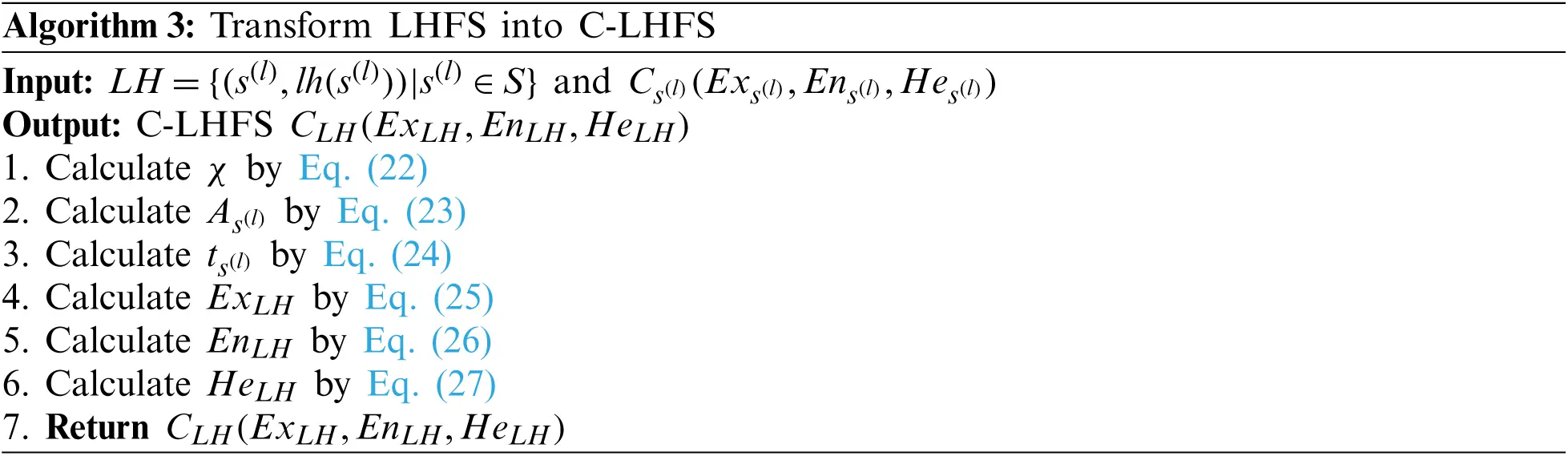Example 5. Given a LTS S={si|i=1,2,,7} and a LHFS LH={(s5,0.6),(s6,0.9)}, C5=(6.1878,0.4672,0.0467) is the corresponding cloud for the LT s5 and C6=(7.8031,0.6354,0.0635) is the corresponding cloud for the LT s6. The C-LHFS CLH(ExLH,EnLH,HeLH) for LH={(s5,0.6),(s6,0.9)} can be obtained as follows:

(1)   Based on Eq. (22), the abscissa value of the intersection point χ is obtained:

Let 0.6×exp((χ5,66.1878)22×0.46722)=0.9×exp((χ5,67.8031)22×0.63542) to solve the abscissa value of the intersection point: χ5,6=6.7966.

(2)   Based on Eq. (23), the area for the NC of C(s5,0.6) from Ex53En5 to the intersection point and the area for the NC of C(s6,0.9) from the intersection point to Ex6+3En6 are obtained:

As5=12π×0.4672×4.78626.7966exp((x6.1878)22×0.46722)dx=0.9024,As6=12π×0.6354×6.79669.7092exp((x7.8031)22×0.63542)dx=0.9421

(3)   Based on Eq. (24), the modified ratios of s5 and s6 are obtained:

ts5=0.90240.9024+0.9421=0.4892,ts6=0.94210.9024+0.9421=0.5108

(4)   Based on Eqs. (25)(27), three numerical characteristics are obtained:

ExLH=0.4892×6.1878+0.5108×7.8031=7.0128,EnLH=0.4892×0.46722+0.5108×0.63542=0.5594,HeLH=0.4892×0.04672+0.5108×0.06352=0.0559

Finally, the C-LHFS of LH={(s5,0.6),(s6,0.9)} is C{ (s5,0.6),(s6,0.9)}=(7.0128,0.5594,0.0559).

The NCs and 5000 cloud drops of C(s5,0.6) and C(s6,0.9) are shown in Fig. 10. The areas for the PDFCs of C(s5,0.6) and C(s6,0.9) are shown in Fig. 11.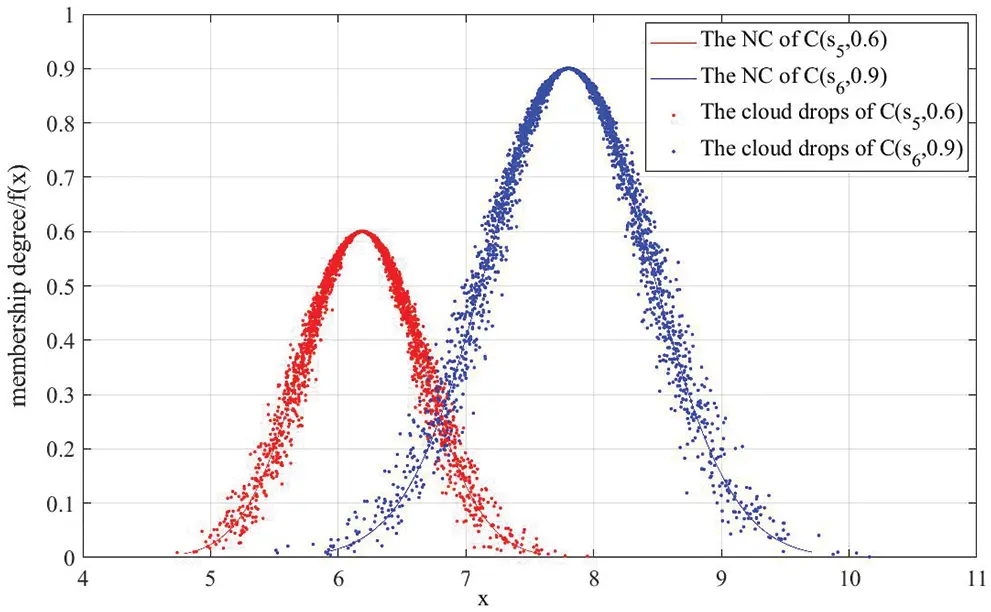Figure 10: NCs and 5000 cloud drops of C(s5,0.6) and C(s6,0.9)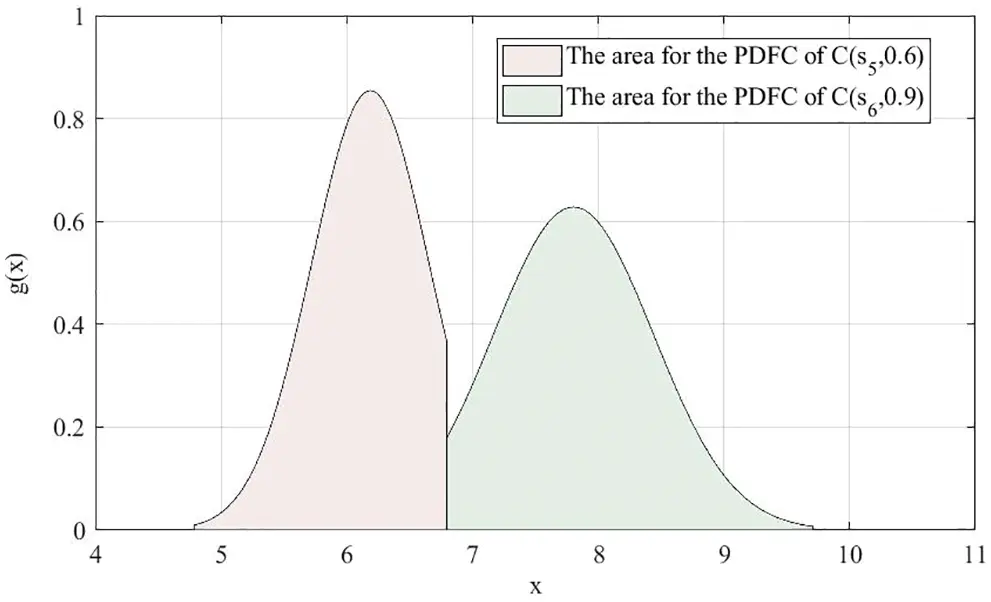Figure 11: Areas for the PDFCs of C(s5,0.6) and C(s6,0.9)

From LH={(s5,0.6),(s6,0.9)}, we can know that the membership degrees of LTs s5 and s6 are 0.6 and 0.9 respectively. If a DM uses LH={(s5,0.6),(s6,0.9)} to evaluate an alternative, it can be assumed that the DM uses 5000 cloud drops to express his/her opinion, then he/she will place 2500 cloud drops in the s5 region and 2500 cloud drops in s6 region. Since the membership degree of LT s5 is 0.6, the maximum membership degree of cloud drops belonging to s5 region should be adjusted to 0.6. For the same reason, the maximum membership degree of cloud drops belonging to s6 region should be adjusted to 0.9. Similar to the process of C-PLTS, parts of the 2500 cloud drops belonging to s5 region will overlap with cloud drops belonging to s6 region, and parts of the 2500 cloud drops belonging to s6 region will overlap with cloud drops belonging to s5 region, which can be seen from Fig. 10. In order to eliminate the information distortion caused by the overlapping part, this paper eliminates the overlapped cloud drops from the cloud drops originally allocated and recalculates the proportions that belong to each region. LHFS contains LTs and their corresponding membership degree. Thus the intersection point between the NC of C(s5,0.6) and the NC of C(s6,0.9) is taken as the boundary to recalculate the proportions of cloud drops distributed in the two regions, which are shown in Fig. 11. From the perspective of membership degree, the C-LHFS is obtained. In the meanwhile, the modified ratios of LTs decrease the loss and distortion of evaluation information.

Up till now, heterogeneous MAGDM matrices in which attribute values are expressed with LTs, PLTSs and LHFSs can be transformed into homogeneous MAGDM cloud matrices. For simplicity, homogeneous MAGDM cloud matrix is called as cloud matrix hereafter. Then, the individual cloud matrix Ce=(Cuve)m×n can be elicited as

Ce=(Cuve)m×n=y1y2ynx1x2xm[C11eC12eC1neC21eC22eC2neCm1eCm2eCmne](e=1,2,,k).(28)

4  Cloud-Based Heterogeneous MAGDM

In this section, some related techniques are introduced, such as the comparison approach for clouds, the determination approaches of DM weight vector and attribute weight vector. Significantly, a personalized comprehensive cloud-based method for heterogeneous MAGDM problem is proposed.

4.1 Determination of DM Weight Vector

As mentioned above, the regulation parameter ς of entropy is determined according to DMs’ hesitant degree and the regulation parameter ζ of hyper entropy is determined according to DMs’ indeterminacy degree and information entropy. Therefore, the larger the two parameters are, the smaller weight should be given to the DM. Assume that there are a series of regulation parameters ςe[1,2] of entropy and a series of regulation parameters ζe[0,1) of hyper entropy for DM de. Based on the regulation parameters ςe and ζe, the weight of DM de can be calculated by

ϖe=3ςeζee=1k(3ςeζe)(e=1,2,,k).(29)

Solving Eq. (29), the DM weight vector v=(ϖ1,ϖ2,,ϖk)T is obtained.

Example 6. Following Example 3, ς1=1, ζ1=0.0352; ς2=1.1470, ζ2=0.2931; ς3=1.1470, ζ3=0.6359.

By Eq. (29), the DM weight vector v=(ϖ1,ϖ2,ϖ3)T=(0.4144,0.3290,0.2567)T is obtained.

Definition 19.  Assume that is the set of all clouds and Ce(Exe,Ene,Hee)(e=1,2,,k) is a subset of . A mapping CWAA: m is defined as the cloud-weighted arithmetic averaging (CWAA) operator so that the following is true:

CWAAv(C1,C2,,Ck)=e=1kϖeCe,(30)

where v=(ϖ1,ϖ2,,ϖk)T is the associated weight vector of Ce(Exe,Ene,Hee) satisfying that 0ϖe1 and e=1kϖe=1.

Based on Eqs. (29) and (30) and basic operations of clouds in Definition 5, the individual cloud matrices Ce=(Cuve)m×n(e=1,2,,k) can be aggregated into a collective cloud matrix Cg=(Cuvg)m×n as

Cg=(Cuvg)m×n=y1y2ynx1x2xm[C11eC12eC1neC21eC22eC2neCm1eCm2eCmne].(31)

4.2 Pairwise Comparisons of Clouds

The evaluations from DMs have been transformed to clouds. As mentioned above, if (x,y) is a cloud drop of C=(Ex,En,He), it is easily known that g=12πEnexp((xEx)22En2) is the PDFC of C. Let C1=(Ex1,En1,He1) and C2=(Ex2,En2,He2) be two clouds, then gC1=12πEn1exp((xEx1)22En12) and gC2=12πEn2exp((xEx2)22En22) are the PDFCs for C1 and C2. GC1(x) and GC2(x) are the corresponding distribution functions respectively. Motivated by the comparison approach for linguistic distributions in , a new comparison approach for clouds is presented in the following.

4.2.1 CASD Relationship

According to the characteristics of cloud, AFSD theory is used to compare the dominance relationship between clouds with characteristics Ex, En in C=(Ex,En,He).

Definition 20. Let Ω={x|GC1(x)>GC2(x)}, Θ={x|GC2(x)>GC1(x)}, and ||GC1(x)GC2(x)||=ΩGC1(x)GC2(x)dx+ΘGC2(x)GC1(x)dx. Let

D21=ΩGC1(x)GC2(x)dx||GC1(x)GC2(x)||.(32)

If D21<0.5, then C1 CASD C2. It is easily seen that D12=1D21. Thus, if D12>0.5, C1 CASD C2 can be obtained as well.

4.2.2 CASD Degree

As mentioned above, the CASD relationship is adapted to compare two clouds. However, this relationship cannot quantify the degree for one cloud over another. To quantify the dominance degree, CASD degree is put forward.

Let μ1 be the threshold for the deviation between Ex1 and Ex2, μ2 be the threshold for the deviation between En1 and En2, and μ3 be the threshold for the deviation between He1 and He2. Let q12 denote the CASD degree for C1 over C2. If C1 CASD C2, q12 can be calculated by dividing into the cases in Table 4. If C1 CASD C2, but Ex1, Ex2, En1, En2, He1 and He2 do not satisfy cases in Table 4, then q12=0.5. If C1 CASD C2 is not verified, then q12=1q21.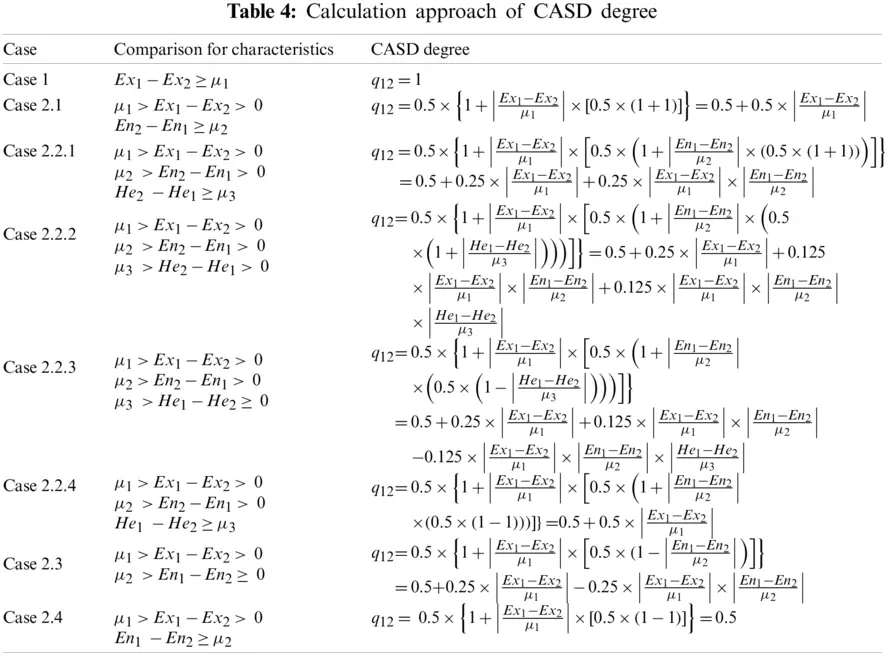To rank the alternatives and select the optimal alternative, the comparison approach for clouds is applied to the collective cloud matrix. Alternatives xu(u=1,2,,m) are compared in pair on each attribute yv(v=1,2,,n). In this paper, the values of μ1, μ2 and μ3 are determined as follows:

μ1=2m(m+1)u=1mo=u+1md(Exu,Exo),(33)

μ2=2m(m+1)u=1mo=u+1md(Enu,Eno),(34)

μ3=2m(m+1)u=1mo=u+1md(Heu,Heo),(35)

Based on the comparison approach mentioned above, the CASD degree for the alternatives xu over xo(u,o=1,2,,m;uo) with respect to attributes yv can be calculated, denoted by quo,v. At the same time, the collective CASD degree matrix Qv=(quo,v)m×m on each attribute yv is obtained:

Qv=(quo,v)m×m=x1x2xmx1x2xm[q12,vq1m,vq21,vq2m,vqm1,vqm2,v](v=1,2,,n).(36)

Let quv denote the collective overall CASD degree for alternatives xu over other alternatives with respect to attribute yv, where

quv=1m1o=1,oumquo,v.(37)

Then, the collective overall CASD degree matrix Q=(quv)m×n can be obtained

Q=(quv)m×n=y1y2ynx1x2xm[q11q12q1nq21q22q2nqm1qm2qmn].(38)

4.3 Determination of Attribute Weight Vector

As mentioned in Section 3.1, the attribute weight vector is denoted by w=(w1,w2,,wn)T, satisfying 0wv1(v=1,2,,n) and v=1nwv=1. In this paper, three perspectives are considered to obtain the attribute weights, which are differentiation between evaluation values, relationship between attributes and the amount of information contained in evaluation values.

4.3.1 From the Perspective of Differentiation between Evaluation Values

Let DEVuv=1m1o=1,oumd(quv,qov) be the deviation between alternative xu and other alternatives on attribute yv, where d(quv,qov)=|quvqov| indicates the distance between quv and qov.

Next, let DEVv=2m(m1)u=1mo=u+1md(quv,qov) be the total deviation of alternative xu on attribute yv.

According to maximizing deviation approach , an attribute with a larger deviation value among alternatives should be assigned a larger weight, and vice versa. Thus, Model 1 is constructed as follows:

Model 1

maxZ1(w)=v=1nDEVvwvs.t.{v=1n(wv)2=1wv0,(v=1,2,,n).(39)

4.3.2 From the Perspective of Relationship between Attributes

Let RELvp=[u=1m(quvqup)]/[u=1m(quv)2u=1m(qup)2] be the correlation coefficient between attribute yv and attribute yp(pv).

Then, let RELv=1n1p=1,pvn[u=1m(quvqup)]/[u=1m(quv)2u=1m(qup)2] be the correlation coefficient between attribute yv and all the other attributes.

From the perspective of correlation coefficient , larger RELv means the elimination of attribute yv has less influence on ordering and attribute yv should be assigned a smaller weight, and vice versa. Based on correlation coefficient, Model 2 is built as follows:

Model 2

maxZ2(w)=v=1n(1RELv)wvs.t.{v=1n(wv)2=1wv0,(v=1,2,,n).(40)

4.3.3 From the Perspective of the Amount of Information Contained in Evaluation Values

Let ENTv=1lnmu=1m(uvlnuv) be the information entropy of attribute yv, where uv=quv/u=1mquv.

It has been mentioned in Section 3.2.2 that information entropy is an important tool to measure the uncertainty of the evaluation information. It is easily known that if the information entropy of evaluations on attribute yv is small, the difference degree contained in evaluations on attribute yv is great, which means the evaluations on attribute yv are informative and attribute yv should be assigned a large weight, and vice versa . Therefore, Model 3 is constructed as follows:

Model 3

maxZ3(w)=v=1n(1ENTv)wvs.t.{v=1n(wv)2=1wv0,(v=1,2,,n).(41)

4.3.4 A Comprehensive Tri-Objective Optimization Model

Combining Eqs. (39)(41), a comprehensive tri-objective optimization model is built as

maxZ1(w)=v=1nDEVvwvmaxZ2(w)=v=1n(1RELv)wvmaxZ3(w)=v=1n(1ENTv)wvs.t.{v=1n(wv)2=1wv0,(v=1,2,,n).(42)

To solve the comprehensive tri-objective optimization model, we add three balance coefficients ψ1, ψ2 and ψ3 into Eq. (42) and convert it into a single-objective optimization model as:

Model 4 {

maxZ(w)=ψ12m(m1)v=1nu=1mo=u+1md(quv,qov)wv+ψ2v=1n(11n1p=1,pvnu=1m(quvqup)u=1m(quv)2u=1m(qup)2)wv+ψ3v=1n(1+1lnmu=1m(uvlnuv))wvs.t.{v=1n(wv)2=1wv0,(v=1,2,,n),(43)

} where ψ1, ψ2 and ψ3 are the balance coefficients, satisfying 0ψ1,ψ2,ψ31 and ψ1+ψ2+ψ3=1. The values of ψ1, ψ2 and ψ3 could be given by DMs in advance, according to the actual situation and personal preference.

To solve Eq. (43), a Lagrange function is constructed as

L(w,λ)=ψ12m(m1)v=1nu=1mo=u+1md(quv,qov)wv+ψ2v=1n(11n1p=1,pvnu=1m(quvqup)u=1m(quv)2u=1m(qup)2)wv+ψ3v=1n(1+1lnmu=1m(uvlnuv))wv+λ2(v=1n(wv)21),(44)

where λ is a real number, denoting the Lagrange multiplier.

The global optimal solution can be derived by taking partial derivatives of wv and λ in Eq. (44), such that

L(w,λ)wv=ψ12m(m1)u=1mo=u+1md(quv,qov)+ψ2(11n1p=1,pvnu=1m(quvqup)u=1m(quv)2u=1m(qup)2)+ψ3(1+1lnmu=1m(uvlnuv))+λwv=0,(45)

L(w,λ)λ=12(v=1n(wv)21)=0.(46)

By solving Eqs. (45) and (46), the solution can be obtained

wv=ψ12m(m1)u=1mo=u+1md(quv,qov)+ψ2(11n1p=1,pvnu=1m(quvqup)u=1m(quv)2u=1m(qup)2)+ψ3(1+1lnmu=1m(uvlnuv))v=1n(ψ12m(m1)u=1mo=u+1md(quv,qov)+ψ2(11n1p=1,pvnu=1m(quvqup)u=1m(quv)2u=1m(qup)2)+ψ3(1+1lnmu=1m(uvlnuv)))2(47)

After normalizing wv(v=1,2,,n) in Eq. (47), we can obtain the attribute weight vector w=(w1,w2,,wn)T, where wv=wv/v=1nwv.

Model 4 enables DMs to make a tradeoff in the above three aspects. Multifaceted considerations enhance the stability of the proposed method and the setting of balance coefficients improves the flexibility of the proposed method.

4.4 Obtaining the Ranking of Alternatives

Up till now, the collective overall CASD degree matrix Q=(quv)m×n and the attribute weight vector w=(w1,w2,,wn)T have been obtained. Thus, the total CASD degree of xu can be calculated as

qu=v=1nquvwv(u=1,2,m).(48)

Based on the values of qu(u=1,2,m), the ranking of alternatives is obtained. The larger qu, the better the alternative xu.

4.5 Decision Steps for the Personalized Comprehensive Cloud-Based Method

A personalized comprehensive cloud-based method for heterogeneous MAGDM problem is proposed in this sub-section. Particularly, the resolution procedures of the proposed method are depicted in Fig. 12.Figure 12: Resolution procedures of the proposed method

As depicted in Fig. 12, the proposed method mainly includes five steps below:

Step 1. Construct the individual original normalized evaluation matrix Re=(ruve)m×n as Eq. (4).

DMs identify the feasible alternatives xu(u=1,2,m) and determine related attributes yv(v=1,2,n) and their evaluation forms, such as LT, PLTS, or LHFS. Each DM gives original evaluation matrix Re=(ruve)m×n. Obtain the individual original normalized evaluation matrix Re=(ruve)m×n by using p~(l)=p(l)/l=1L(p)p(l), where

Re=(ruve)m×n={si,ifyvisbenefitattributeneg(si),ifyviscostattribute{s(l)(p(l))|s(l)S},ifyvisbenefitattribute{neg(s(l))(p(l))|s(l)S},ifyviscostattribute{(s(l),lh(s(l)))|s(l)S},ifyvisbenefitattribute{(neg(s(l)),lh(s(l)))|s(l)S},ifyviscostattribute.

Step 2. Transform the individual original normalized evaluation matrix Re=(ruve)m×n to the individual cloud matrix Ce=(Cuve)m×n as Eq. (28).

Hesitant degree HDe, information entropy He and indeterminacy degree IDe for DM de are calculated according to the individual original evaluation matrix Re=(ruve)m×n based on Eqs. (5), (6) and (8)(11). Then, the regulation parameters of entropy and hyper entropy for each DM are calculated by Eqs. (7) and (12), respectively. LTs, PLTSs and LHFSs are transformed into clouds, C-PLTSs and C-LHFSs by the improved transformation approaches in Sections 3.2 and 3.3, respectively.

Step 3. Aggregate the individual cloud matrices Ce=(Cuve)m×n(e=1,2,,k) into the collective cloud matrix Cg=(Cuvg)m×n as Eq. (31) with basic operations of clouds and CWAA operator.

Based on Eq. (29), DM weight vector v=(ϖ1,ϖ2,,ϖk)T can be acquired according to the regulation parameters ςe and ζe of each DM.

Step 4. Transform the collective cloud matrix Cg=(Cuvg)m×n to the collective overall CASD degree matrix Q=(quv)m×n as Eq. (38).

Firstly, pairwise comparisons are made to judge the CASD relationships for alternatives xu(u=1,2,m) on each attribute yv according to Eq. (32). Then, the thresholds for Ex, En and He are calculated based on Eqs. (33)(35) and the CASD degrees are calculated according to Table 4. At the same time, the collective CASD degree matrices Qv=(quo,v)m×m(v=1,2,,n) on different attributes yv(v=1,2,,n) are obtained, as Eq. (36). Finally, calculate the collective overall CASD degrees quv for alternatives xu(u=1,2,m) over other alternatives on each attribute yv by Eq. (37) and generate the collective overall CASD degree matrix Q=(quv)m×n.

Step 5. Generate the ranking order of all alternatives xu according to the decreasing order of the total CASD degrees qu(u=1,2,m).

Set the balance coefficients ψ1, ψ2, ψ3 for Eq. (43). After obtaining the attribute weight vector w=(w1,w2,,wn)T by Model 4, the total CASD degrees of xu(u=1,2,m) can be calculated by Eq. (48). Based on the values of qu(u=1,2,m), the ranking of alternatives is obtained.

5  Illustrative Example

In this section, the proposed method is applied to an example of emergency medical waste disposal site selection in COVID-19. Furthermore, sensitivity analyses are conduced to demonstrate the stability and flexibility of the proposed method.

5.1 Illustration of the Proposed Method

5.1.1 Background Description

At the end of 2019, COVID-19 broke out in various provinces and cities in China. The amount of medical waste kept rising along with the number of confirmed cases. The explosive growth of medical waste occurred in many cities, and the lack of disposal capacity made the situation more serious. In such an emergency situation, medical waste disposal becomes a special battlefield in the fight against pneumonia. If these massive amounts of medical waste that may carry the virus were not disposed in a safe and timely way, it was likely to cause secondary infections and further spread of COVID-19, which may result in a series of unimaginable aftermaths. Generally, qualified medical waste disposal companies existed previously were completely at full capacity in many cities during the outbreak of COVID-19. In order to cope with the increasing amount of medical waste, many local governments adopted a series of emergency measures. One of these measures was converting other waste disposal companies, such as industrial hazardous waste disposal companies and solid waste disposal companies, to medical waste disposal sites temporarily for emergency disposal of medical waste. The selection for emergency medical waste disposal sites can be regarded as a heterogeneous MAGDM problem.

To select a suitable emergency medical waste disposal site from five alternatives {x1,x2,x3,x4,x5}, a panel of four experts {d1,d2,d3,d4} were appointed to evaluate the five alternatives on five attributes: geographical location (y1), equipment (y2), process technologies (y3), disposal capacity (y4) and transport capacity (y5). The five attributes all are qualitative benefit attributes. The LTS is predefined as S={s1:verybad;s2:bad;s3:alittlebad;s4:medium;s5:alittlegood;s6:good;s7:verygood}. The evaluations for geographical location (y1) can be evaluated by LTs. PLTSs are used to evaluate equipment (y2) and process technologies (y3). LHFSs are used to evaluate disposal capacity (y4) and transport capacity (y5). The evaluations of all alternatives on the five attributes given by the four DMs are normalized and listed in Table 5.

5.1.2 Resolution Process by Using the Proposed Method of This Paper

The procedures are summarized in the following steps:

Step 1. The individual original normalized evaluation matrices Re=(ruve)5×5(e=1,2,3,4) have been obtained and presented in Table 5.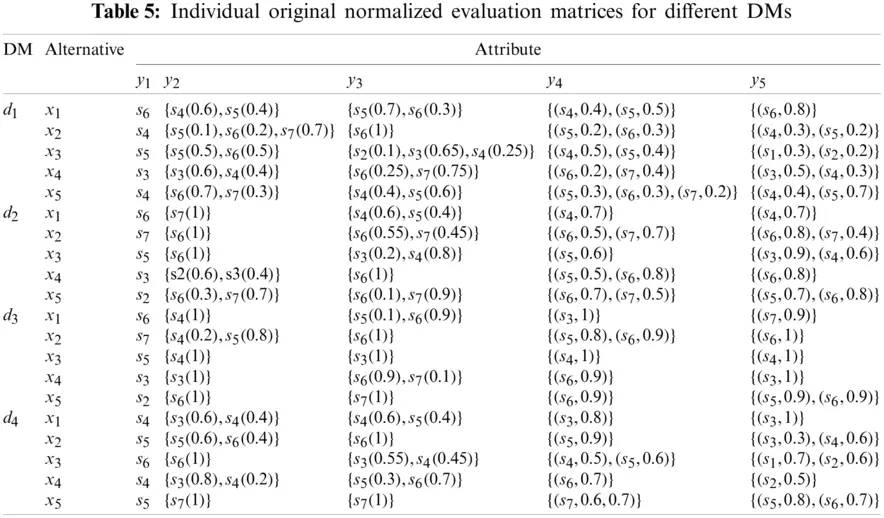Step 2. Based on Eqs. (5)(12), Hesitant degree HD, information entropy H, indeterminacy degree ID, regulation parameters ς and ζ for each DM are calculated and presented in Table 6.According to the proposed transformation approaches from LTs, PLTSs and LHFSs to clouds, C-PLTSs and C-LHFSs, the individual original normalized evaluation matrices Re=(ruve)5×5(e=1,2,3,4) have been transformed into individual cloud matrices Ce=(Cuve)5×5(e=1,2,3,4). LSF2 Eq. (2) and a=1.36 are adopted in this paper. All the individual cloud matrices are listed in Table 7.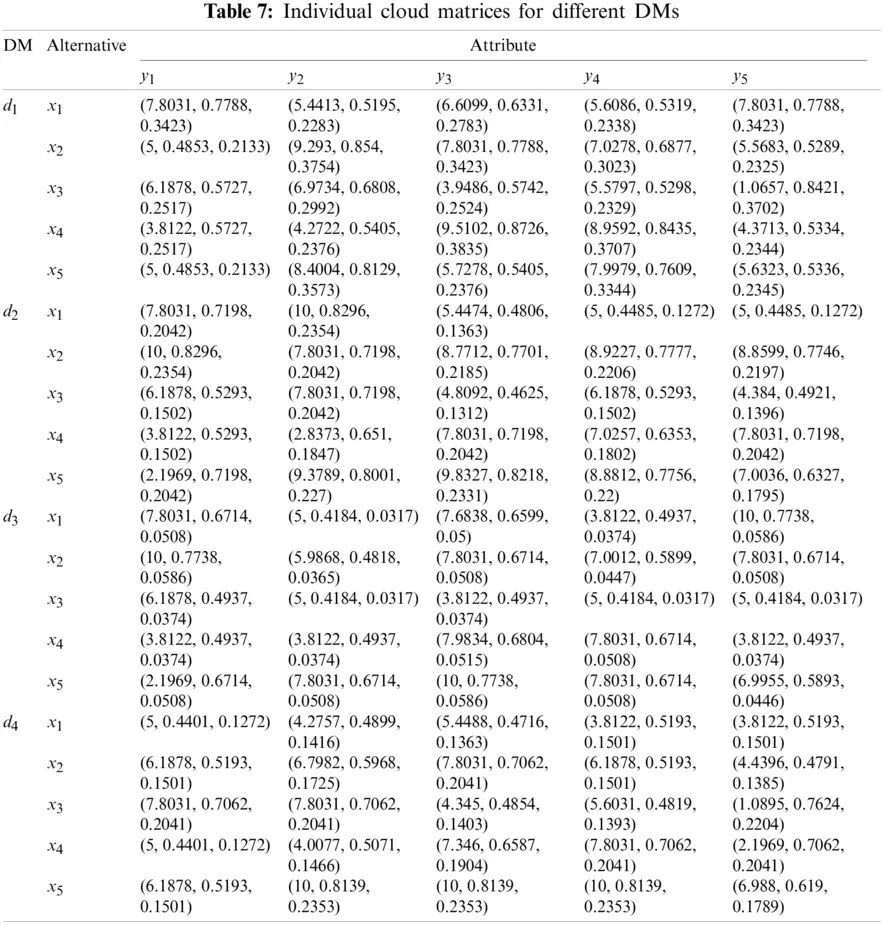Step 3. DMs’ weights are calculated by Eq. (29). With basic operations of clouds, CWAA operator, and DM weight vector v=(ϖ1,ϖ2,ϖ3,ϖ4)T=(0.1989,0.2488,0.3,0.2523)T, the collective cloud matrix Cg=(Cuvg)5×5 is obtained and shown in Table 8.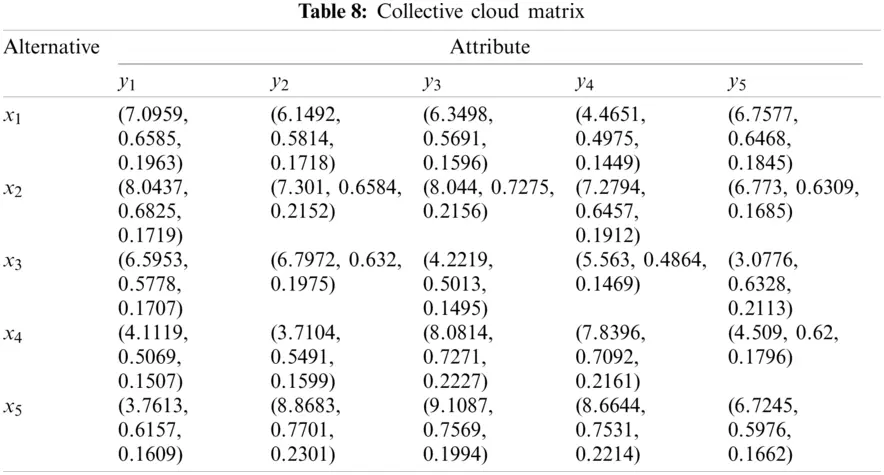Step 4. Compare the alternatives xu(u=1,2,5) in pair and determinate the CASD relationships on each attribute yv by Eq. (32). The CASD degrees are calculated according to Eqs. (33)(35) and Table 4. The collective CASD degree matrices Qv=(quo,v)5×5(v=1,2,5) are shown in Table 9. Subsequently, the collective overall CASD degrees for alternative xu(u=1,2,5) over other alternatives on each attribute yv are calculated by Eq. (37). The collective overall CASD degree matrix Q=(quv)5×5 is presented in Table 10.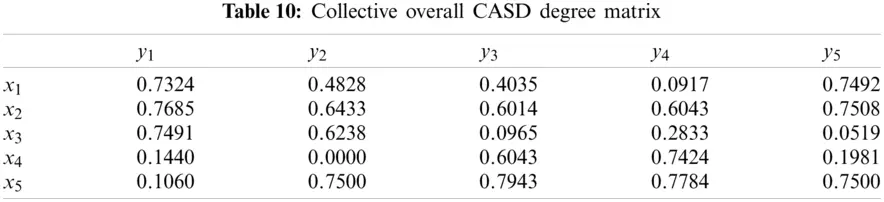Step 5. Balance coefficients ψ1=13, ψ2=13, ψ3=13 are set for Eq. (43) in this example. By Model 4, the attribute weight vector is obtained as w=(w1,w2,w3,w4,w5)T=(0.2368,0.1886,0.1664,0.2036,0.2046)T.

With the obtained attribute weight vector, the total CASD degrees of qu(u=1,2,5) are calculated by Eq. (48) and the results are listed as follows:

q1=0.5036,q2=0.68,q3=0.3794,q4=0.3263,q5=0.6107

Thus, the ranking order is x2x5x1x3x4 and x2 is the optimal alternative.

5.2 Sensitivity Analyses

LSFs are strictly monotonously increasing with respect to the subscript i. In linguistic evaluation scales, the absolute deviation of semantics between any two adjacent LTs may increase, decrease or remain unchanged with increasing linguistic subscripts. DMs can choose different LSFs according to the actual situation and personal preference. In Eq. (43), ψ1, ψ2, ψ3 are considered as the balance coefficients for each perspective of attribute weights obtaining, satisfying 0ψ1,ψ2,ψ31 and ψ1+ψ2+ψ3=1. The values of ψ1, ψ2, ψ3 are given by DMs in advance. This sub-section takes different LSFs and different balance coefficients to solve the above example. The corresponding decision results are listed in Table 11 and shown in Fig. 13. The average differences of total CASD degrees between two adjacent alternatives in ranking results are shown in Fig. 14.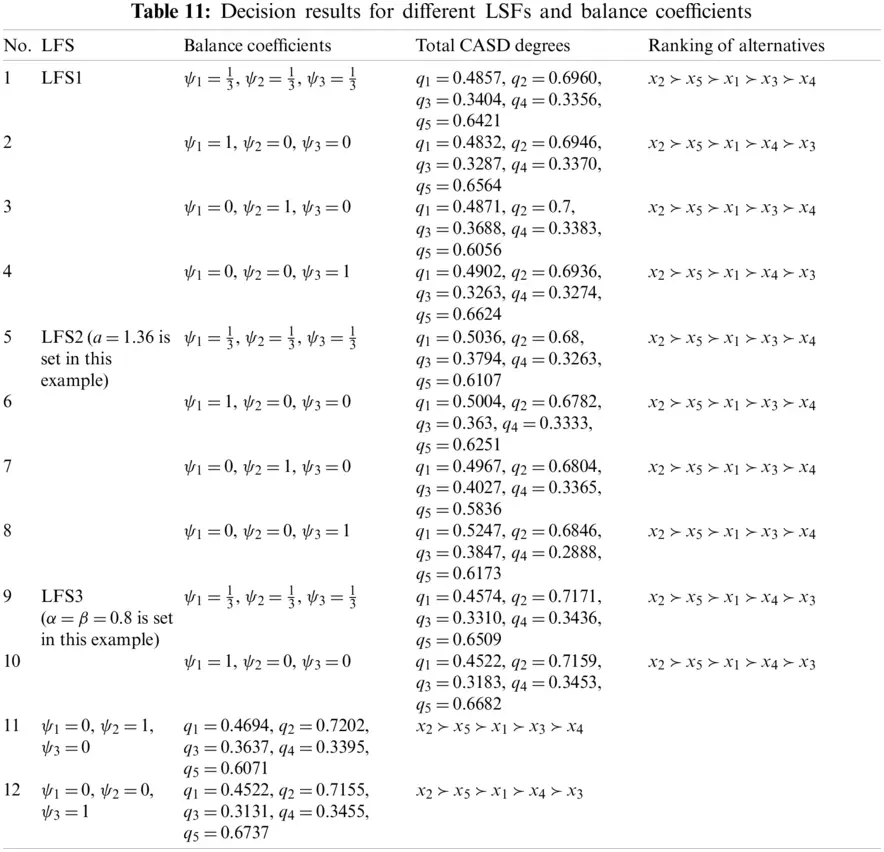Figure 13: Demonstration of ranking results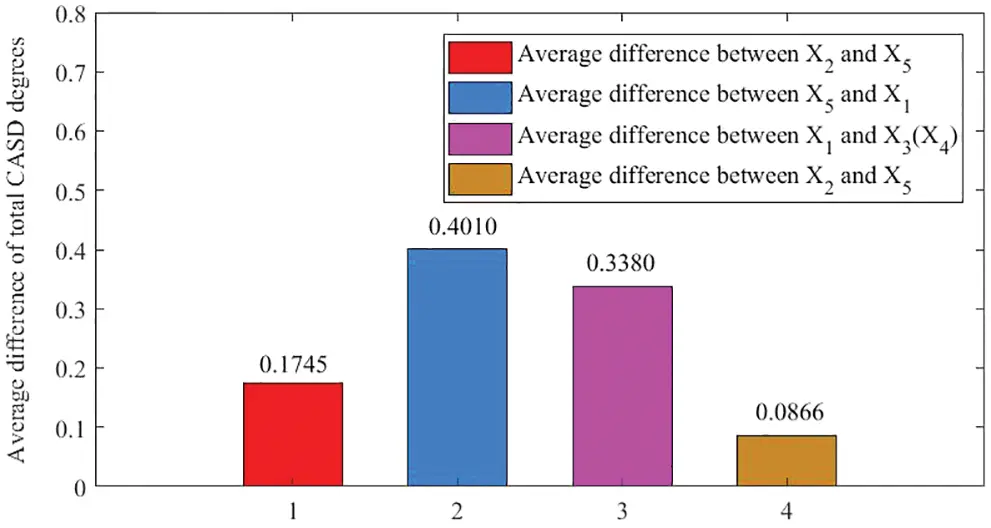Figure 14: Average differences of total CASD degrees

As can be seen from Table 11 and Fig. 13, the ranking order of alternatives is x2x5x1x3x4 or x2x5x1x4x3. Besides, it is easy to discover from Table 11 that the top three alternatives are always x2, x5 and x1, which indicates that the alteration of LSFs and balance coefficients has only little impact on the ranking order of the alternatives. Therefore, the proposed method has high stability in determining the optimal alternative.

Furthermore, the proposed method can handle various decision situations and meet different DMs’ preferences by taking different LSFs and balance coefficients. Thus, the flexibility of the proposed method can be reflected by the acquired ranking results derived by various selections of LSFs and balance coefficients.

6  Comparison Analyses and Discussion

To justify the advantages of our proposal, comparison analyses with methods based on cloud and other classical MAGDM methods are conducted. Besides, a summary of transformation approaches with different evaluation forms is presented.

6.1 Comparison with Methods Based on Cloud

Peng et al.  proposed a new method based on cloud to handle MAGDM problems with PLTSs. Hu  proposed two methods based on comprehensive cloud aggregation operator to solve MAGDM problems with LHFSs. This paper proposes a novel method based on cloud for heterogeneous MAGDM, which could handle MAGDM problems with LT, PLTS, LHFS or one of them. Obviously, the aforesaid methods all are based on cloud. The proposed method could handle MAGDM problems in [23,32], while Peng et al.'s method  and Hu's methods  could not solve the problem of this paper. Thus, the proposed method has wider applicability. Except for wider applicability, other important distinguishing factors and superiorities of the proposed method are stated as follows:

(1)   The cloud in  contains five characteristics, yet the values of left and right entropy are averaged into one in this paper, which greatly reduce the complexity of the following calculation. The transformation from LTs to clouds in  is based on the golden radio, while it is based on LSFs in this paper. The selection for LSFs and its related parameters makes the transformation more flexible and practical. In addition, this paper proposes regulation parameters for entropy and hyper entropy. DMs’ personalities can be reflected with the incorporation of regulation parameters in the transformation from LTs to clouds. Moreover, the modified ratios of LTs decrease the loss and distortion of evaluation information in the transformation from PLTSs (LHFSs) to C-PLTSs (C-LHFSs).

(2)   The method in  determines the attribute weights only via maximizing deviation, while three perspectives are considered to obtain the attribute weights in this paper. Besides, the setting of balance coefficients enhances the flexibility of the proposed method. Moreover, three steps are needed to obtain attribute weights in , including determining the individual weights of criteria, determining the weights associated with groups (equivalent to DMs in this paper) and calculating the overall weights of attributes. By contrast, only one step is needed to obtain attribute weights by the proposed method, which reduces the complexity of the calculation greatly.

(3)   The proposed approach to determining DMs’ weights is superior to . Hu  and this paper both consider the number of LTs and corresponding indeterminacy degree in LHFS. However, if all the DMs only use one LT with different memberships in all LHFSs, the determination approach of DMs’ weights in  becomes invalid. For example, d1 and d2 give their evaluation matrices as follows:

R1=y1y2x1x2[ (s6,1)(s3,0.9)(s3,1)(s1,0.95) ]R2=y1y2x1x2[ (s5,0.1)(s3,0.2)(s4,0.05)(s1,0.1) ]

It can be seen that d1 and d2 does not have the same confidence for their own evaluation matrices, but they will be assigned the same weight in . However, d1 and d2 will be assigned different weights in this paper for their different membership for LTs. Obviously, the determination approach of DMs’ weights in this paper is more reasonable.

(4)   The ranking of alternatives is based on the expected score values of clouds in . The expected score values of clouds sometimes are unstable and may lead to inaccurate decision results. By contrast, the ranking of alternatives is based on the total CASD degree in this paper. Obviously, the ranking approach of this paper is more stable.

6.2 Comparison with Other Classical MAGDM Methods

Lin et al.  put forward two novel TOPSIS-ScoreC-PLTS and VIKOR-ScoreC-PLTS methods to handle MAGDM problems with PLTSs. This paper proposes a personalized comprehensive cloud-based method for heterogeneous MAGDM, which could handle MAGDM problems with LT, PLTS, LHFS or one of them. To justify the advantages of our proposal, comparison analyses with Lin et al.'s method  are conducted as follows:

(1)   Solve the adapted example of this paper by the methods in 

Since Lin et al.'s method just cloud handle the MAGDM problems with PLTSs, we only retain the evaluations on y1, y2 and y3 in the site selection example of emergency medical waste disposal and replace Eq. (12) by ζe=logρ2(He+1). The adapted problem is dealt with the TOPSIS-ScoreC-PLTS method in , the VIKOR-ScoreC-PLTS method in  and the proposed method, respectively. The ranking results are displayed in Table 12.It is easy to find that x2 is always the optimal alternative and x4 is always the worst alternative, which shows the effectiveness of the proposed method.

(2)   Solve the example in  by the proposed method of this paper

The proposed method could handle MAGDM problems with LT, PLTS, LHFS or one of them. As a result, the proposed method could settle the example in  directly and the calculation results are as follows:

DM weight vector: v=(ϖ1,ϖ2,ϖ3,ϖ4)T=(0.2415,0.2399,0.2545,0.2641)T.

Attribute weight vector: w=(w1,w2,w3,w4,w5)T=(0.2071,0.2331,0.1871,0.2205,0.1523)T.

The total CASD degrees: q1=0.6808, q2=0.4606, q3=0.3932, q4=0.4654.

Therefore, the final ranking order is x1x4x2x3 and x1 is the optimal alternative.

The ranking order by method in  is x1x2x3x4 and x1x3x2x4. No matter by method in  or the proposed method, x1 is the best alternative. However, the ranking of the rest alternatives differs greatly. Obviously, the most remarkable difference is the ranking order of x4. After analysis, it is easily found that equal weights are given to each DM directly in , while higher weights are given to DMs with more informative evaluations in this paper. DMs d3 and d4 are given higher weights for their informative evaluations and they give overwhelming evaluations to x4 on y1. Thus, the ranking of x4 improves a lot by the proposed method. It is clear that the proposed method of this paper is more objective and practical.

6.3 Comparison with Other Transformation Approaches

Previous studies [2227,3034] have proposed a lot of transformation approaches from LT and its’ extended forms to cloud and comprehensive clouds. A specific summary is shown in Table 13.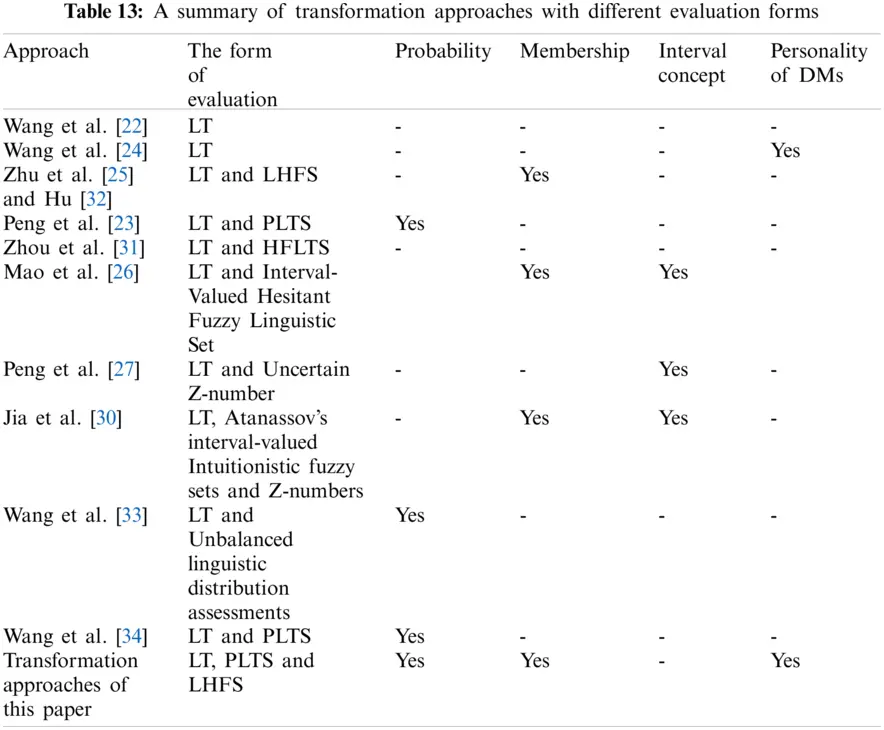In summary, we find that most existing studies can only process LTs, or LTs with probability, or LTs with membership or LTs with interval concept. However, this paper provides the transformation approaches for LTs, LTs with probability and LTs with membership, simultaneously. Moreover, there are few studies that take DMs’ personalities into account during the transformation process. Although Wang et al.  introduced overlap parameter into the transformation process to reflect the DMs’ personality, the determination of overlap parameter is a little subjective. This paper proposes regulation parameters for entropy and hyper entropy and further incorporates them into the transformation process from LTs to clouds to reflect the different personalities of DMs. It is worth emphasizing that the determination of regulation parameters is totally objective. Apparently, the proposed transformation approaches of this paper are more applicable and effective.

7  Conclusion

This paper develops a personalized comprehensive cloud-based method for heterogeneous MAGDM, in which the evaluations of alternatives on attributes are represented as LTs, PLTSs and LHFSs. The validity of the proposed method is demonstrated with a site selection example of emergency medical waste disposal in COVID-19. The effectiveness, stability, flexibility and superiorities of the proposed method are proven by sensitivity and comparison analyses, respectively. Compared with the existing methods, the proposed method of this paper has the following prominent superiorities:

(1)   With the proposed regulation parameters, the width and thickness of clouds for the corresponding LTS are different for different DMs, which makes the DMs’ personalities can be reflected in clouds. Besides, a novel approach to obtaining DM weight vector is constructed based on the proposed regulation parameters.

(2)   The new transformation approaches from PLTS and LHFS to C-PLTS and C-LHFS decrease the loss and distortion of evaluation information.

(3)   CASD relationship and CASD degree are initiated in this paper to compare clouds. With CASD relationship and CASD degree, alternatives in the form of clouds can be ranked and the ranking results are stable and effective. This innovation provides new perspective for pairwise comparisons of clouds.

(4)   The comprehensive tri-objective programing constructed in this paper enables DMs to make a tradeoff among three different aspects. Multifaceted considerations enhance the stability of the proposed method and the setting of balance coefficients improves the flexibility of the proposed method.

Although an example of emergency medical waste disposal site selection in COVID-19 is illustrated to the effectiveness of the proposed method, and it is expected to be applied to more real-life decision-making problems, such as investment selection, supply chain management, and so on. More effective transformation approaches for other evaluation forms, especially LTs with interval concept are waiting for us to come up with and apply them to heterogeneous MAGDM problems. Additionally, how to extend some classical decision-making methods to heterogeneous MAGDM based on cloud is also very interesting and deserves to be studied in the future.

Funding Statement: This research was supported by the National Natural Science Foundation of China (Nos. 62141302, 11861034 and 71964014), the Humanities Social Science Programming Project of Ministry of Education of China (No. 20YJA630059), the Natural Science Foundation of Jiangxi Province of China (No. 20212BAB201011), and the Postgraduate Innovation Fund Project of Jiangxi Province (No. YC2020-S290).

Conflicts of Interest: The authors declare that they have no conflicts of interest to report regarding the present study.

## References

1. Yu, S. M., Du, Z. J., Wang, J. Q., Luo, H. Y., Lin, X. D. (2021). Trust and behavior analysis-based fusion method for heterogeneous multiple attribute group decision-making. Computers & Industrial Engineering, 152(8), 106992. DOI 10.1016/j.cie.2020.106992.
2. Boran, F. E., Genç, S., Kurt, M., Akay, D. (2009). A Multi-attributes intuitionistic fuzzy group decision making for supplier selection with TOPSIS method. Expert Systems with Applications, 36(8), 11363–11368. DOI 10.1016/j.eswa.2009.03.039.
3. Liang, R. X., Wang, J. Q., Li, L. (2018). Multi-attributes group decision-making method based on interdependent inputs of single-valued trapezoidal neutrosophic information. Neural Computing & Applications, 30(1), 241–260. DOI 10.1007/s00521-016-2672-2.
4. Qin, J. D., Liu, X. W., Pedrycz, W. (2017). An extended TODIM multi-attributes group decision making method for green supplier selection in interval type-2 fuzzy environment. European Journal of Operational Research, 258, 626–638. DOI 10.1016/j.ejor.2016.09.059.
5. Wang, J. Q., Peng, J. J., Zhang, H. Y., Liu, T., Chen, X. H. (2015). An uncertain linguistic multi-attributes group decision-making method based on a cloud model. Group Decision and Negotiation, 24(1), 171–192. DOI 10.1007/s10726-014-9385-7.
6. Tian, Z. P., Wang, J., Wang, J. Q., Zhang, H. Y. (2017). Simplified neutrosophic linguistic multi-attributes group decision-making approach to green product development. Group Decision and Negotiation, 26(3), 597–627. DOI 10.1007/s10726-016-9479-5.
7. Pang, Q., Wang, H., Xu, Z. (2016). Probabilistic linguistic term sets in multi-attribute group decision making. Information Sciences, 369, 128–143. DOI 10.1016/j.ins.2016.06.021.
8. Meng, F., Chen, X., Zhang, Q. (2014). Multi-attribute decision analysis under a linguistic hesitant fuzzy environment. Information Sciences, 267, 287–305. DOI 10.1016/j.ins.2014.02.012.
9. Liu, A. H., Wan, S. P., Dong, J. Y. (2021). An axiomatic design-based mathematical programming method for heterogeneous multi-criteria group decision making with linguistic fuzzy truth degrees. Information Sciences, 571, 649–675. DOI 10.1016/j.ins.2021.04.091.
10. Gao, Y., Li, D. S. (2019). A consensus model for heterogeneous multi-attribute group decision making with several attribute sets. Expert Systems with Applications, 125, 69–80. DOI 1016/j.eswa.2019.01.072.
11. Wan, S. P., Zou, W. C., Dong, J. Y. (2020). Prospect theory based method for heterogeneous group decision making with hybrid truth degrees of alternative comparisons. Computers & Industrial Engineering, 141, 106285. DOI 10.1016/j.cie.2020.106285.
12. Merigo, J. M., Palacios-Marques, D., Zeng, S. Z. (2016). Subjective and objective information in linguistic multi-criteria group decision making. European Journal of Operational Research, 248(2), 522–531. DOI 10.1016/j.ejor.2015.06.063.
13. Merigo, J. M., Gil-Lafuente, A. M. (2013). Induced 2-tuple linguistic generalized aggregation operators and their application in decision-making. Information Sciences, 236, 1–16. DOI 10.1016/j.ins.2002.039.
14. Li, D. Y., Liu, C., Gan, W. (2009). A new cognitive model: Cloud model. Wiley Subscription Services, 24(3), 357–375. DOI 10.1002/int.20340.
15. Li, D. Y., Du, Y. (2007). Artificial intelligent with uncertainty. China: Journal of Software.
16. Zhang, H. Y., Ji, P., Wang, J. Q., Chen, X. H. (2016). A neutrosophic normal cloud and its application in decision making. Cognitive Computation, 8, 649–669. DOI 10.1007/s12559-016-9394-8.
17. Li, D. Y. (1997). Knowledge representation in KDD based on linguistic atoms. Journal of Computer Science and Technology, 12(6), 481–496. DOI 10.1007/BF02947201.
18. Li, D., Han, J., Shi, X., Man, C. (1998). Knowledge representation and discovery based on linguistic atoms. Knowledge-Based Systems, 10(7), 431–440. DOI 10.1016/S0950-7051(98)00038-0.
19. Guan, X. J., Qian, L., Li, M. X., Zhou, L. G. (2017). Earthquake relief emergency logistics capacity evaluation model integrating cloud generalized information aggregation operators. Journal of Intelligent & Fuzzy Systems, 32(3), 2281–2294. DOI 10.3233/JIFS-16252.
20. Deng, W. H., Wang, G. Y., Xu, J. (2016). Piecewise two-dimensional normal cloud representation for time-series data mining. Information Sciences, 374, 32–50. DOI 10.1016/j.ins.2016.09.027.
21. Di, K. C., Li, D. Y., Li, D. R. (1999). Cloud theory and its applications in spatial data mining and knowledge discovery. Journal of Image and Graphics, 11(4), 930–935. DOI 10.3969/j.issn.1006-8961.1999.11.006.
22. Wang, J. Q., Peng, L., Zhang, H. Y., Chen, X. H. (2014). Method of multi-attribute group decision-making based on cloud aggregation operators with linguistic information. Information Sciences, 274, 177–191. DOI 10.1016/j.ins.2014.02.130.
23. Peng, H. G., Zhang, H. Y., Wang, J. Q. (2018). Cloud decision support model for selecting hotels on tripadvisor.com with probabilistic linguistic information. International Journal of Hospitality Management, 68, 124–138. DOI 10.1016/j.ijhm.2017.10.001.
24. Wang, P., Xu, X., Cai, C., Huang, S. (2018). A linguistic large group decision making method based on the cloud model. IEEE Transactions on Fuzzy Systems, 26(6), 3314–3326. DOI 10.1109/TFUZZ.2018.2822242.
25. Zhu, C. X., Zhu, L., Zhang, X. Z. (2016). Linguistic hesitant fuzzy power aggregation operators and their applications in multiple attribute decision-making. Information Sciences, 367, 809–826. DOI 10.1016/j.ins.2016.07.011.
26. Mao, X. B., Hu, S. S., Dong, J. Y., Wan, S. P., Xu, G. L. (2018). Multi-attribute group decision making based on cloud aggregation operators under interval-valued hesitant fuzzy linguistic environment. International Journal of Fuzzy Systems, 20(7), 2273–2300. DOI 10.1007/s40815-018-0495-2.
27. Peng, H. G., Zhang, H. Y., Wang, J. Q., Li, L. (2019). An uncertain z-number multicriteria group decision-making method with cloud models. Information Sciences, 501, 136–154. DOI 10.1016/j.ins.2019.05.090.
28. Song, W., Zhu, J. (2019). A multistage risk decision making method for normal cloud model considering behavior characteristics. Applied Soft Computing Journal, 78, 393–406. DOI 10.1016/j.asoc.2019.02.033.
29. Wang, P., Zhang, J., Zhang, W. W. (2021). Multi-granularity linguistic large group decision-making based on cloud model and multi-layer weight determination. Control and Decision, 36(9), 2257–2266. DOI 10.13195/j.kzyjc.2020.0102.
30. Jia, Q., Hu, J., He, Q., Zhang, W., Safwat, E. (2021). A multicriteria group decision-making method based on AIVIFSs, z-numbers, and trapezium clouds. Information Sciences, 566, 38–56. DOI 10.1016/j.ins.2021.02.042.
31. Zhou, J., Liu, L. F., Sun, L. J., Xiao, F. (2018). A Multi-criteria decision-making method for hesitant fuzzy linguistic term set based on the cloud model and evidence theory. Journal of Intelligent & Fuzzy Systems, 36(2), 1–12. DOI 10.3233/JIFS-18051.
32. Hu, S. S. (2018). Multi-attribute group decision making based on comprehensive cloud under linguistic hesitant fuzzy environment and its application in ERP selection (Master Thesis). Jiangxi University of Finance and Economics, China.
33. Wang, X. K., Wang, Y. T., Zhang, H. Y., Wang, J. Q., Li, L. et al. (2021). An asymmetric trapezoidal cloud-based linguistic group decision-making method under unbalanced linguistic distribution assessments. Computers & Industrial Engineering, 160, 107457. DOI 10.1016/j.cie.2021.107457.
34. Wang, X. K., Wang, S. H., Zhang, H. Y., Wang, J. Q., Li, L. (2021). The recommendation method for hotel selection under traveller preference characteristics: A cloud-based multi-criteria group decision support model. Group Decision and Negotiation, 30, 1433–1469. DOI 10.1007/s10726-021-09735-0.
35. Xu, Z. S. (2005). Deviation measures of linguistic preference relations in group decision making. Omega, 33(3), 249–254. DOI 10.1016/j.omega.2004.04.008.
36. Wang, J. Q., Peng, H. G., Zhang, H. Y., Wang, J. (2017). An extended outranking approach for multi-criteria decision-making problems with linguistic intuitionistic fuzzy numbers. Applied Soft Computing, 59, 462–474. DOI 10.1016/j.asoc.2017.06.013.
37. Bao, G. Y., Lian, X. L., He, M., Wang, L. L. (2010). Improved two-tuple linguistic representation model based on new linguistic evaluation scale. Control and Decision, 25(5), 780–784. DOI 10.3724/SP.J.1087.2010.02828.
38. Tversky, A., Kahneman, D. (1992). Advances in prospect theory: Cumulative representation of uncertainty. Journal of Risk and Uncertainty, 5(4), 297–323. DOI 10.1007/BF00122574.
39. Li, D. Y., Meng, H. J., Shi, X. M. (1995). Membership clouds and membership cloud generator. Journal of Computer Research and Development, 32(6), 15–20.
40. Qin, K., Kai, X., Liu, F. L., Li, D. Y. (2011). Image segmentation based on histogram analysis utilizing the cloud model. Computers & Mathematics with Applications, 62(7), 2824–2833. DOI 10.1016/j.camwa.2011.07.048.
41. Schmid, F., Trede, M. (1996). Testing for first-order stochastic dominance: A New distribution-free test. Journal of the Royal Statistical Society: Series D (The Statistician), 45(3), 371–380.
42. Chen, X., Liang, H. M., Gao, Y., Xu, W. J. (2020). A method based on the disappointment almost stochastic dominance degree for the multi-attribute decision making with linguistic distributions. Information Fusion, 54, 10–20. DOI 10.1016/j.inffus.2019.06.027.
43. Wan, S. P., Li, D. F. (2013). Fuzzy LINMAP approach to heterogeneous MADM considering comparisons of alternatives with hesitation degrees. Omega, 41(6), 925–940. DOI 10.1016/j.omega.2012.12.002.
44. Wang, H. L., Feng, Y. Q. (2005). On multiple attribute group decision making with linguistic assessment information based on cloud model. Control and Decision, 20(6), 679–685. DOI 10.13195/j.cd.2005.06.79.wanghl.017.
45. Wang, Y., Tian, L., Wu, Z. (2021). Trust modeling based on probabilistic linguistic term sets and the MULTIMOORA method. Expert Systems with Applications, 165(4), 113817. DOI 10.1016/j.eswa.2020.113817.
46. Zhao, K., Gao, J. W., Qi, Z. Q., Li, C. B. (2015). Multi-criteria risky decision-making approach based on prospect theory and cloud model. Control and Decision, 30(3), 395–402. DOI 10.13195/j.kzyjc.2013.1773.
47. Wang, Y. (1997). Using the method of maximizing deviation to make decision for multi-indices. Journal of Systems Engineering and Electronics, 8(3), 21–26.
48. Liu, X. D., Zhu, J. J., Zhang, S. T., Liu, G. D. (2016). Hesitant fuzzy multiple attribute decision making method based on optimization of attribute weights. Control and Decision, 31(2), 297–302. DOI 10.13195/j.kzyjc.2014.1931.
49. Xu, Z. S., Xia, M. M. (2012). Hesitant fuzzy entropy and cross-entropy and their use in multi-attribute decision-making. International Journal of Intelligent Systems, 27(9), 799–822. DOI 10.1002/int.21548.
50. Lin, M. W., Chen, Z. Y., Xu, Z. S., Gou, X. J., Herrera, F. (2021). Score function based on concentration degree for probabilistic linguistic term sets: an application to TOPSIS and VIKOR. Information Sciences, 551, 270–290. DOI 10.1016/j.ins.2020.10.061.This work is licensed under a Creative Commons Attribution 4.0 International License, which permits unrestricted use, distribution, and reproduction in any medium, provided the original work is properly cited.